5.2.3 Excitation coding

26.4453GPPCodec for Enhanced Voice Services (EVS)Detailed algorithmic descriptionRelease 15TS

The excitation signal coding depends on the coding mode. In general it can be stated that in the absence of DTX/CNG operation, the excitation signal is coded per subframes of 64 samples. This means that it is encoded four times per frame in case of 12.8 kHz internal sampling rate and five times per frame in case of 16 kHz internal sampling rate. The exception is the GSC coding where longer subframes can be used to encode some components of the excitation signal, especially at lower bitrates.

The excitation coding will be described in the following subclauses, separately for each coding mode. The description of excitation coding starts with the GC and VC modes. For the UC, TC, and GSC modes, it will be described in subsequent subclauses with references to this subclause.

5.2.3.1 Excitation coding in the GC, VC and high rate IC/UC modes

The GC,VC and high rate IC/UC modes are very similar and are described together. The VC mode is used in stable voiced segments where the pitch is evolving smoothly within an allowed range as described in subclause 5.1.13.2. Thus, the major difference between the VC and GC modes is that more bits are assigned to the algebraic codebook and less to the adaptive codebook in case of the VC mode as the pitch is not allowed to evolve rapidly in the VC mode. The high-rate IC and UC modes are similar and are used for signalling inactive frames where only a background noise is detected, and unvoiced frames, respectively. The two modes differ from GC mode mainly by their specific gain coding codebook. The GC mode is then used in frames not assigned to a specific coding mode during the signal classification procedure and is aimed at coding generic speech and audio frames. The principle of excitation coding is shown in a schematic diagram in figure 15. The individual blocks and operations are described in detail in the following subclauses.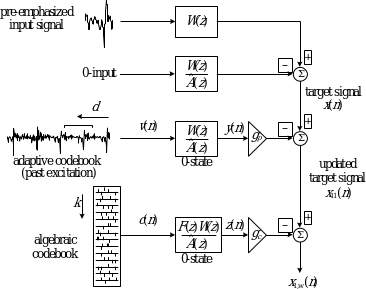Figure 25: Schematic diagram of the excitation coding in GC and VC mode

5.2.3.1.1 Computation of the LP residual signal

To keep the processing flow similar for all coding modes, the LP residual signal is computed for the whole frame in the first processed subframe of each frame, as this is needed in the TC mode. For each subframe, the LP residual is given by

(497)

where is the pre-emphasized input signal, defined in subclause 5.1.4 and are the quantized LP filter coefficients, described in subclause 5.2.2.1.

In DTX operation the computed LP residual signalis attenuated by multiplying an attenuation factorfor all input bandwidths except NB. The attenuation factor is calculated as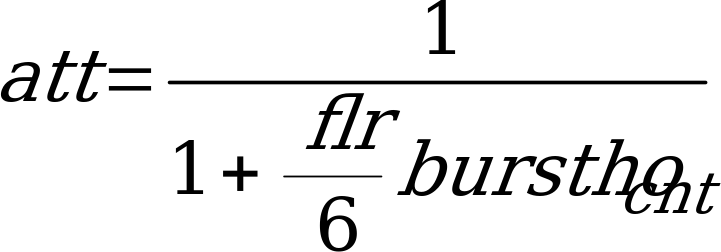(497a)

where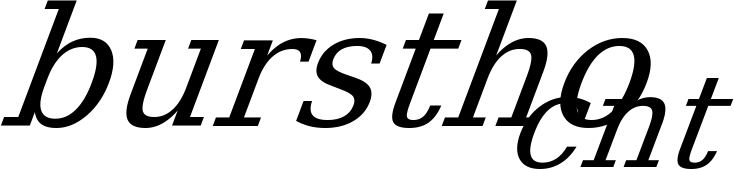as determined in subclause 5.6.2.1.1 is upper limited by,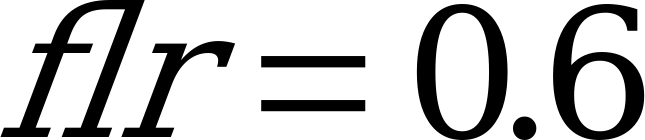if the bandwidth is not WB or the latest bitrate used for actively encoded framesis larger than 16.4 kbps. Otherwiseis determined from a hangover attenuation table as defined in Table 35b.is only updated in the first SID frame after an active signal period if two criteria are both fulfilled. The first criterion is satisfied if AMR-WB IO mode is used or the bandwidth=WB. The second criterion is met if the number of consecutive active frames in the latest active signal segment was at least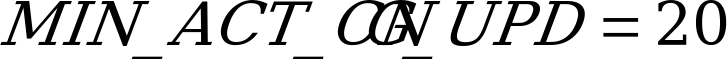number of frames or if the current SID is the very first encoded SID frame. The attenuation factoris finally lower limited to.

Table 35b: Attenuation floor

 Latest active bitrate [kbps]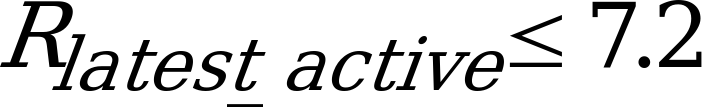0.53703180.61659500.6839116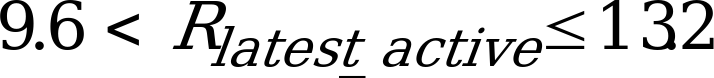0.7079458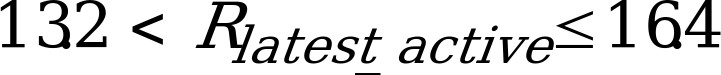0.7079458
5.2.3.1.2 Target signal computation

The target signal for adaptive codebook search is usually computed by subtracting a zero-input response of the weighted synthesis filter from the weighted pre-emphasized input signal. This is performed on a subframe basis. An equivalent procedure for computing the target signal, which is used in this codec, is filtering of the residual signal, , through the combination of the synthesis filter and the weighting filter. After determining the excitation signal for a given subframe, the initial states of these filters are updated by filtering the difference between the LP residual signal and the excitation signal. The memory update of these filters is explained in subclause 5.2.3.1.8. The residual signal, , which is needed for finding the target vector, is also used in the adaptive codebook search to extend the past excitation buffer. This simplifies the adaptive codebook search procedure for delays less than the subframe size of 64 as will be explained in the next subclause. The target signal in a given subframe is denoted as .

5.2.3.1.3 Impulse response computation

The impulse response, , of the weighted synthesis filter

(498)

is computed for each subframe. Note that is not the impulse response of the filter, but of the filter . In the equation above,, is the quantized LP filter, the coefficients of which are (see subclause 5.2.2.1). This impulse response is needed for the search of adaptive and algebraic codebooks. The impulse responseis computed by filtering the vector of coefficients of the filter, extended by zeros, through the two filters: and.

The adaptive codebook search consists of performing a closed-loop pitch search, and then computing the adaptive codevector, , by interpolating the past excitation at the selected fractional pitch lag. The adaptive codebook parameters (or pitch parameters) are the closed-loop pitch, , and the pitch gain, (adaptive codebook gain), calculated for each subframe. In the search stage, the excitation signal is extended by the LP residual signal to simplify the closed-loop search. The adaptive codebook search is performed on a subframe basis. The bit allocation is different for the different modes.

In the first and third subframes of a GC, UC or IC frame, the fractional pitch lag is searched with a resolution in the range [34, 91½], and with integer sample resolution in the range [92, 231]depending on the bit-rate and coding mode. Closed-loop pitch analysis is performed around the open-loop pitch estimates. Always bounded by the minimum and maximum pitch period limits, the range [–8, +7] is searched in the first subframe, while the range [–8, +7] is searched in the third subframe. The pitch period quantization limits are summarized in table 46.

Table 36: Pitch period quantization limits

 Rates (kbps) Sampling rate of the limits (kHz) IC/UC VC GC 7.2 12.8 n.a. [17; 231] [34; 231] 8.0 12.8 n.a. [17; 231] [20; 231] 9.6 12.8 n.a. [29; 231] [29; 231] 13.2 12.8 n.a. [17; 231] [20; 231] 16.4 16 n.a. [36; 289] [36; 289] 24.4 16 n.a. [36; 289] [36; 289] 32 16 [21; 289] n.a. [21; 289] 64 16 [21; 289] n.a. [21; 289]

For the second and fourth subframes, a pitch resolution depending on the bit-rate and coding mode is used and the closed-loop pitch analysis is performed around the closed-loop pitch estimates, selected in the preceding (first or third) subframe. If the closed-loop pitch fraction in the preceding subframe is 0, the pitch is searched in the range [–8, +7½], whereis the integer part of the fractional pitch lag of the preceding subframe (p is either 0, to denote the first subframe, or 3 to denote the third subframe). If the fraction of the pitch in the previous subframe is , the pitch is searched in the range [–7, +8½]. The pitch delay is encoded as follows. In the first and third subframe, absolute values of the closed-loop pitch lags are encoded. In the third and fourth subframe, only relative values with respect to the absolute ones are encoded.

In the VC mode, the closed-loop pitch lag is encoded absolutely in the first subframe and relatively in the following 3 subframes. If the fraction of the closed-loop pitch of the preceding subframe is 0, the pitch is searched in the interval [–4, +3½]. If the fraction of the closed-loop pitch lag in the preceding subframe is , the pitch is searched in the range [–3, +4½].

The closed-loop pitch search is performed by minimizing a mean-squared weighted error between the target signal and the past filtered excitation (past excitation, convolved with). This is achieved by maximizing the following correlation

(499)

where is the target signal andis the past filtered excitation at delay k. Note that negative indices refer to the past signal. Note also that the search range is limited around the open loop pitch lags, as explained earlier. The convolution of the past excitation signal withis computed only for the first delay in the searched range. For other delays, it is updated using the recursive relation

(500)

where , , is the excitation buffer. Note that in the search stage, the samples, , are unknown and they are needed for pitch delays less than 64. To simplify the search, the LP residual signal, , is copied tofor , in order to make the relation in equation (501) valid for all delays. If the optimum integer pitch lag is in the range [34, 91], the fractions around that integer value are tested. The fractional pitch search is performed by interpolating the normalized correlation of equation (502) and searching for its maximum. The interpolation is performed using an FIR filter for interpolating the term in equation (503) using a Hamming windowed sinc function truncated at. The filter has its cut off frequency (–3 dB) at 5050 Hz and –6 dB at 5760 Hz in the down-sampled domain, which means that the interpolation filter exhibits low-pass frequency response. Note that the fraction is not searched if the selected best integer pitch coincides with the lower end of the searched interval.

Once the fraction is determined, the initial adaptive codevector, , is computed by interpolating the past excitation signal at the given phase (fraction). In the following text, the fractional pitch lags (not the fractions) in all subframes will be denoted as, where the index denotes the subframe.

In order to enhance the coding performance, a low-pass filter can be applied to the adaptive codevector. This is important since the periodicity doesn’t necessarily extend over the whole spectrum. The low pass filter is of the form . Thus, the adaptive codevector is given by

(504)

where for for rates at and above 32kbps and otherwise.

An adaptive selection is possible by sending 1 bit per sub-frame. There are then two possibilities to generate the excitation, the adaptive codebook , in the first path, or its low pass-filtered version as described above in the second path. The path which results in minimum energy of the target signal is selected for the filtered adaptive codebook vector.

Alternatively, the first or the second path can be used without any adaptive selection. Table 37 summarizes the strategy for the different combinations.

Table 38: Adaptive codebook filtering configuration

 Rates (kbps) IC/UC VC GC 7.2 n.a. Non-filtered LP filtered 8.0 n.a. Non-filtered LP filtered 9.6 n.a. Non-filtered LP filtered 13.2 n.a. Adaptive selection Adaptive selection 16.4 LP-filtered Adaptive selection LP filtered 24.4 LP-filtered Adaptive selection LP filtered 32 n.a. n.a. Adaptive selection 64 n.a. n.a. Adaptive selection

5.2.3.1.4.2 Computation of adaptive codevector gain

The adaptive codevector gain (pitch gain) is then found by

(505)

where is the filtered adaptive codevector (zero-state response of to).

To avoid instability in case of channel errors, is limited by 0.95, if the pitch gains of the previous subframes have been close to 1 and the LP filters of the previous subframes have been close to being unstable (highly resonant).

The instability elimination method tests two conditions: resonance condition using the LP spectral parameters (minimum distance between adjacent LSFs), and gain condition by testing for high valued pitch gains in the previous frames. The method works as follows. First, a minimum distance between adjacent LSFs is computed as…

At 9.6, 16.4 and 24.4 kbps, the gain is further constrained. It is done for helping the recovery after the loss of a previous frame.

(506)

5.2.3.1.5 Algebraic codebook

An important feature of this codebook is that it is a dynamic codebook, whereby the algebraic codevectors are filtered through an adaptive pre-filter. The transfer function of the adaptive pre-filter varies in time in relation to parameters representative of spectral characteristics of the signal. The pre-filter is used to shape the frequency characteristics of the excitation signal to damp frequencies perceptually annoying to the human ear. Here, a pre-filter relevant to WB signals is used which consists of two parts: a periodicity enhancement part and a tilt part . That is,

(507)

The periodicity enhancement part of the filter colours the spectrum by damping inter-harmonic frequencies, which are annoying to the human ear in case of voiced signals. T is the integer part of the closed-loop pitch lag in a given subframe (representing the fine spectral structure of the speech signal) rounded to the ceiling, i.e.,, where i denotes the subframe.

The factorof the tilt part of the pre-filter is related to the voicing of the previous subframe. At 16.4 and 24.4 kbps it is bounded by [0.28, 0.56] and it computed as

(508)

Otherwise it is bounded by [0.0, 0.5] and is given by

(509)

whereand are the energies of the scaled pitch codevector and the scaled algebraic codevector of the previous subframe, respectively. The role of the tilt part is to reduce the excitation energy at low frequencies in case of voiced frames.

Depending on bitrates, coding mode and the estimated level of background noise, the adaptive pre-filter also includes a filter based on the spectral envelope, which colours the spectrum by damping frequencies between the formant regions. The final form of the adaptive pre filter is given by

(510)

where and if Hz and and if Hz.

The codebook search is performed in the algebraic domain by combining the pre-filter, , with the weighted synthesis filter prior to the codebook search. Thus, the impulse response of the weighted synthesis filter must be modified to include the pre-filter . That is, , where is the impulse response of the pre-filter.

5.2.3.1.5.2 Overview of Algebraic codebooks used in EVS

Depending on the bitrate and rendered bandwidth, algebraic codebooks of different sizes are used in the EVS codec. The following tables summarize the codebooks used in each subframe at different bitrates of the EVS codec

Table 38: NB Algebraic codebook configurations (bits/subframe)

 Rate (kbps) IC UC VC GC 7.2 n.a. n.a. 12/12/12/20 12/12/12/20 8.0 n.a. n.a. 12/20/12/20 12/20/12/20 9.6 30/32/32/32 30/32/32/32 28/28/28/28 24/26/24/26 13.2 n.a. n.a. 36/43/36/43 36/36/36/43 16.4 56/58/56/58 56/58/56/58 56/56/56/58 55/56/55/56 24.4 96/98/96/98 96/98/96/98 96/96/96/98 94/96/96/96

Table 39: WB Algebraic codebook configurations (bits/subframe)

 Rate (kbps) IC UC VC GC VC-FEC GC-FEC GSC 7.2 n.a. n.a. 12/12/12/20 12/12/12/20 n.a. n.a. n.a. 8.0 n.a. n.a. 12/20/12/20 12/20/12/20 n.a. n.a. n.a. 9.6 28/28/28/28 28/28/28/28 26/26/26/28 20/26/24/24 n.a. n.a. n.a. 13.2 n.a. n.a. 28/36/36/36(TD BWE)36/36/36/43(FD BWE) 28/36/28/36(TD BWE)36/36/36/36(FD BWE) n.a. n.a. n.a. 16.4 43/43/43/43/43 43/43/43/43/43 40/43/43/43/43 40/43/40/43/43 n.a. n.a. n.a. 24.4 75/75/75/75/75 75/75/75/75/75 73/75/73/75/75 73/73/73/75/73 73/73/73/73/75 70/75/73/73/73 n.a. 32 12/12/12/12/12 n.a. n.a. 36/36/36/36/36 n.a. n.a. n.a. 64 12/12/12/12/12 n.a. n.a. 36/36/36/36/36 n.a. n.a. n.a.

Table 40: SWB Algebraic codebook configurations (bits/subframe)

 Rate (kbps) IC UC VC GC VC-FEC GC-FEC GSC 9.6 24/26/24/26 24/26/24/26 20/26/24/24 20/20/20/20 n.a. n.a. n.a. 13.2 n.a. n.a. 28/36/28/36 28/28/28/36 n.a. n.a. n.a. 16.4 36/36/36/36/36 36/36/36/36/36 34/36/36/36/36 34/36/34/36/36 n.a. n.a. n.a. 24.4 62/65/62/65/62 62/65/62/65/62 62/62/62/65/62 62/62/62/62/62 62/62/62/62/62 61/61/62/61/62 n.a. 32 12/12/12/12/12 n.a. n.a. 36/28/28/36/36 n.a. n.a. n.a. 64 12/12/12/12/12 n.a. n.a. 36/36/36/36/36 n.a. n.a. n.a.

Table 41: FB Algebraic codebook configurations (bits/subframe)

 Rate (kbps) IC UC VC GC VC-FEC GC-FEC 16.4 36/36/36/36/36 36/36/36/36/36 34/36/36/34/36 34/34/36/34/36 n.a. n.a. 24.4 62/62/65/62/65 62/62/65/62/65 62/62/62/62/62 62/62/62/62/62 61/62/61/62/62 61/61/61/61/61 32 12/12/12/12/12 n.a. n.a. 36/28/28/36/36 n.a. n.a. 64 12/12/12/12/12 n.a. n.a. 36/36/36/36/36 n.a. n.a.

VC-FEC and GC-FEC are specific configurations for which 4 bits are reserved to transmit LPC-based information exploited by the decoder in case of error of the previous frame.

5.2.3.1.5.3 Codebook structure and pulse indexing of the 7-bit codebook

In the 7-bit codebook, the algebraic vector contains only 1 non-zero pulse at one of 64 positions. The pulse position is encoded with 6 bits and the sign of the pulse is encoded with 1 bit. This gives a total of 7 bits for the algebraic code. The sign index here is set to 1 for positive signs and 0 for negative signs.

5.2.3.1.5.4 Codebook structure and pulse indexing of the 12-bit codebook

In the 12-bit codebook, the algebraic vector contains only 2 non-zero pulses. The 64 positions in a subframe are divided into 2 tracks, where each track contains one pulse, as shown in table 39.

Table 40: Potential positions of individual pulses in the 12-bit algebraic codebook

 Track Pulse Positions 1 0 0, 2, 4, 6, 8, 10, 12, 14, 16, 18, 20, 22, 24, 26, 28, 30, 32, 34, 36, 38, 40, 42, 44, 46, 48, 50, 52, 54, 56, 58, 60, 62 2 1 1, 3, 5, 7, 9, 11, 13, 15, 17, 19, 21, 23, 25, 27, 29, 31, 33, 35, 37, 39, 41, 43, 45, 47, 49, 51, 53, 55, 57, 59, 61, 63

Each pulse position in one track is encoded with 5 bits and the sign of the pulse in the track is encoded with 1 bit. This gives a total of 12 bits for the algebraic code. The sign index here is set to 0 for positive signs and 1 for negative signs.

The index of the signed pulse is given by

(511)

where is the position index, is the sign index, and is the number of bits per track. For example, a pulse at position 31 has a position index of 31/2 = 15 and it belongs to the track with index 1 (second track).

5.2.3.1.5.5 Codebook structure and pulse indexing of the 20-bit and larger codebooks

In the 20-bit or larger codebooks, the codevector contains 4 non-zero pulses. All pulses can have the amplitudes +1 or –1. The 64 positions in a subframe are divided into 4 tracks, where each track contains one pulse, as shown in table 41.

Table 43: Potential positions of individual pulses in the 20-bit algebraic codebook

 Track Pulse Positions 1 0 0, 4, 8, 12, 16, 20, 24, 28, 32 36, 40, 44, 48, 52, 56, 60 2 1 1, 5, 9, 13, 17, 21, 25, 29, 33, 37, 41, 45, 49, 53, 57, 61 3 2 2, 6, 10, 14, 18, 22, 26, 30, 34, 38, 42, 46, 50, 54, 58, 62 4 3 3, 7, 11, 15, 19, 23, 27, 31, 35, 39, 43, 47, 51, 55, 59, 63

Each pulse position in one track is encoded with 4 bits and the sign of the pulse in the track is encoded with 1 bit. This gives a total of 20 bits for the algebraic code.

5.2.3.1.5.6 Pulse indexing of the algebraic codebook

The objective is to enumerate all possible constellations of pulses in a vector which corresponds to one track of length within a sub-frame. That is, vector has signed integer values such that its norm-1 is , whereby we say that contains pulses.

We can then partition the vector into two parts, such that the partitions are of length and and contain and pulses respectively. The number of different constellations for the original vector can then be determined by the recursive formulae:

(512)

For computational efficiency, the values of this function can be pre-calculated and placed in a table.

Above equation gives the number of possible states for given and . We can then enumerate a specific state, where and have and pulses respectively. The number of states that have less pulses than in partition is

(513)

We can then define that overall state has , whereby the overall state can be encoded with the recursion

(514)

where the boundary conditions are

(515)

The state can be decoded by the algorithm

1. Set and choose partitioning length and .
2. Calculate with .
3. If then . Otherwise, set and go to 2.

The states of the partitions and can then be calculated from the integer and reminder parts of the fraction We can then recursively determine the state of each position in the vector until a partition has , whereby

(516)

Observe that both the number of states as well as the state are integer numbers which can become larger than 32 bits. We must therefore employ arithmetic operations which support long integers throughout the algorithm.

5.2.3.1.5.7 Pulse indexing of the 43-bit codebook

The joint indexing encoding procedure of three pulses on two tracks is described as follows:

For 3 pulses on a track, the occurrence probability of 3 different pulse positions on a track is the highest, and the occurrence probability of 2 different pulse positions on a track is the second highest, and even the pulses have a higher occurrence probability on the left position of the track than on the right position of the track because the algebraic codebooks need to compensate for the boundary leap of adaptive codebooks between two neighbour sub-frame. So the case of the first pulse with lower position order will be encoded with a smaller index value and the case of more different pulse positions with higher occurrence probability will also be encoded with a smaller index value. The rule is same in case of more than 3 pulses on a track. This rule can be used to save bit in the multi-track joint indexing encoding.

1. Firstly, the pulse information for each track is indexed as follows: (here we suppose that pulses are assigned for each track, and the total quantity of positions on the track is )
2. Analyse the statistics about the positions of the pulses to be encoded on a track and obtain pulse distribution on the track, it includes: quantity (namely ) of pulse positions with pulses in it, the pulse position distribution which includes pulse position vector: , is the quantity of pulse positions , is the ith pulse positions with pulse in it on the track, and quantity of pulses in each pulse position with pulse in it which includes pulse number vector , , where is the number of pulses per track, is the number of pulses in position , and pulse sign vector , is the ith sign in position . If there are pulses having the same positions (pulses with the same positions have the same signs), they are merged into one pulse and the number of pulses for each pulse position as well as the pulse sign is saved. Pulse position are sorted in ascend order, the pulse sign is also adjusted based on the order of pulse position.
3. Compute the offset index according to the quantity of pulse positions, the offset index is saved in a table and used in both encoder and decoder sides. Each offset index in the table indicate a unique number of pulse positions in the track, in case , the offset index only indicate a pulse distribution of pulse positions on the track , in case , the offset index indicate many which have a same pulse distribution of pulse positions on the track .
4. Compute the pulse- position index according to the pulse distribution of pulse positions on the track (). The only indicate a pulse distribution of pulse positions on the track among all the pulse distribution of . Permuting serial numbers of the positions and all possible values of are ordered from a smaller value to a greater value , refers to the quantity of positions with pulses in it, is the total quantity of positions on the track. Compute by using the permutation method as follows:

(514)

wherein represents a position serial number of an nth position that has pulses on it, , , , . For 43bit mode, 3 pulses on a track, , .

(515)

Compute the pulse-number index according to the quantity of pulses in each pulse position as follows:

is determined according to which represents the quantity of pulses in each position with pulses. In order to determine correspondence between and through algebraic calculation, a calculation method of the third index is provided below:

For a track, situations that a track with pulse positions and pulses are mapped to situations that a positions track have pulses, where represents the total number of pulses that are required to be encoded and on the track. For example, in the condition of 6-pulse 4-position ( =6, =4) situations, is {1, 2, 1, 2}, 1 is subtracted from the number of pulses in each position (because each position has at least one pulse) to obtain {0, 1, 0, 1}, that is, information of is mapped to a 2-pulse 4-position ( =2, =4) encoding situation. Figure 16 gives an example of the mapping for .Figure 17: Example of mapping for

According to set order, all possible distribution situations of pulses on positions are arrayed, and an arrayed serial number is used as the index indicating the number of pulses on a position that has pulse.

A calculation formula reflecting the foregoing calculation method is:

(517)

wherein , represents a position serial number of an (h + 1)th pulse, , , , and ∑ indicates summation.

The pulse sign represented by may be a positive value or a negative value. A simple coding mode is generally applied, represents a positive pulse and represents a negative pulse.

Generate the global index . Combine the indices , , and to get the global index as follows :

(518)

(519)

Here is the upper range of which is also the number of total permutations of pulses.

2. Combine the index of the two 3-pulse tracks together which is encoded as in step 1, suppose the indexes of the two tracks are and respectively, and , then the is as below:

(520)

3. Encode the joint index . (Suppose encode with 25 bits). In order to reduce the number of bits used for pulse indexing, a threshold is set at 3611648 for 3-pulses, according to the the pulse number, combination of the occurrence probability and the number of bits that may be saved. If the joint index is smaller than , 24 bits will be used to encode the joint index . If the joint index is bigger than or equal to , will be added into the joint index and 25 bits will be used to encode the joint index . This procedure is described as below:

If (<)

{

is encoded with 24 bits.

}

Else

{

is encoded with 25 bits.

}

For two pulses on the other track, the index for each track is encoded just as pulse indexing of the 20-bit codebook, but there is no joint indexing procedure, then the index for each track is transmitted one by one.

5.2.3.1.5.8 Multi-track joint coding of pulse indexing

The codebook for more than three pulses on a track have idle space in difference ratio, joint encoding for more than two tracks may enable idle codebook spaces in single-track encoding to be combined, and once combined idle spaces are sufficient, one actual encoding bit may be reduced. If several encoding indexes are directly combined, the final encoding length may be very large, or even may exceed the bit width (such as 64 bits) generally used for operating, so a general solution is to split each encoding index into two part and only all the high part is combined together in order to avoid directly combining.

The method is described as follows: the value range of the original index is divided into several intervals by a factor , correspondingly the original index is split into two indexes and by the factor , the length of each interval is not greater than , is a positive integer, denotes a serial number of an interval to which belongs, and denotes a serial number of in the interval to which belongs (apparently,), and: ;

The most economical case of splitting is performed as following:

, where denotes rounding down to an integer, and

, where denotes taking a remainder.

If a combined index needs to achieve better effect of saving encoding bits, it is needed to select a split index that retains the space characteristics of as much as possible, and therefore, for the track t providing a split index to participate in combination,

if , it is appropriate to select Indt0 to participate in combination, and

if , it is appropriate to select Indt1 to participate in combination.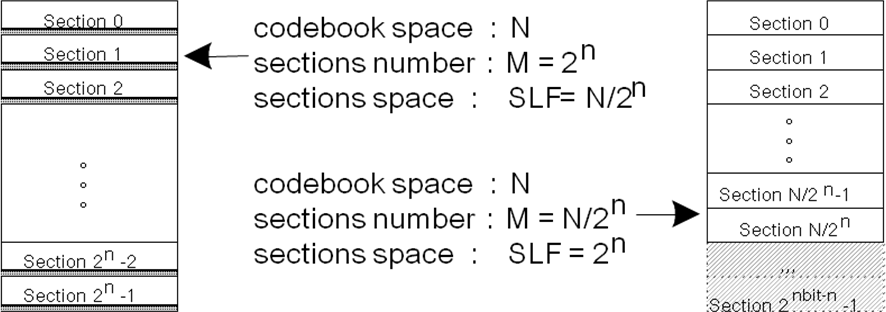Figure 18: The split factor selection and the corresponding codebook space section

Each track may adopt different , according to the pulse number on it

Table 42: the parameters for multi-track joint coding

 pulse bits Codebook space Hi Bit effective ratio re-back bits Dec Hex value bits range 8bit 16bit 24bit 1 5 32 2 9 512 200 1 1 2 1.00 0 3 13 5472 1560 A8 8 172 0.6875 3 4 16 44032 AC00 AC 9 345 0.67578125 1 9 5 19 285088 459A0 8B 8 140 0.546875 5 6 21 1549824 17A600 BD 8 190 0.7421875 3 7 23 7288544 6F36E0 DE 8 223 0.875 1 9 8 25 30316544 1CE9800 1CE 9 463 0.904297 8 9 27 113461024 6C34720 6C3 11 1732 0.845704 8

Multi-track joint coding processing is described as following:

Calculate an encoding index of each track, (subscript t denotes the tth track), split into two split indexes and according to a set factor combine a split index of each track to generate a combined index . The combined index is split into recombined indexes according to the re-back bits length, and each recombined index and an un-combined split index of a corresponding track are respectively combined, then obtain the final recombined index with fixed length 8,16 or 24 bits.

For 4 track in a sub-frame, the algebraic codebook 94bit(8777)~108bit(9999) use 24 bits mode joint en/decoding，the algebraic codebook 62bit(4444)~92bit(7777) use 16 bits mode joint en/decoding，the algebraic codebook 40bit(3222)~61bit(4443) use 8 bits mode joint en/decoding.

All the encoding steps are described as following:

1. Get the parameter from table 43 according to the pulse number of each track, include the index , , , . And get the 8/16/24 mode also according to the pulse number of all track.
2. The index of is split into and , the is , and length of is , length of is ,
3. Combine the and into as following:

(521)

1. Split the low part of and get the , and the length of is ,which get from table 44 in step 1, the and are combine into a with the length of 8,16 or 24 bits.
2. The high part of continue combining with the next as following:

(522)

1. Split the low part of and get the , and the length of is ,which get from table 45 in step 1, the and are combine into a with the length of 8,16 or 24 bits.
2. The high part of continue combining with the next as following:

(523)

1. Split the low part of and get the , and the length of is ,which get from table 46 in step 1, the and are combine into a with the length of 8,16 or 24 bits.
2. The high part of is split into two parts. The low part of is used as the , and the length of is which is obtained from table 47 in step 1, the and are combined into a with the length of 8,16 or 24 bits.
3. Finally, the high part of together with, , and are the outputs of multi-track joint coding and stored into the stream in 16 bits unit.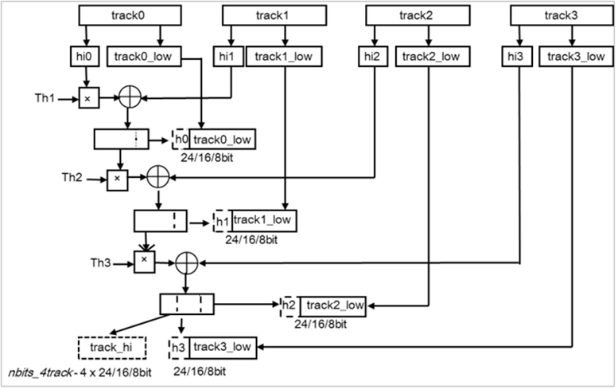Figure 19: Schematic diagram of 4-track joint coding

5.2.3.1.5.9 The search criterion at lower bitrates

The algebraic codebook is searched by minimizing the error between an updated target signal and a scaled filtered algebraic codevector. The updated target signal is given by

(524)

where is the filtered adaptive codevector and is the unquantized adaptive codebook gain. Thus, the updated target signal is obtained by subtracting the adaptive contribution from the initial target signal, .

Let a matrix be defined as a lower triangular Toeplitz convolution matrix with the main diagonal and lower diagonals , and (also known as the backward filtered target vector) be the correlation between the updated signal and the impulse response . Furthermore, let be the matrix of correlations of . Here, is the impulse response of the combination of the synthesis filter, the weighting filter and the pre-filter which includes a long-term filter.

The elements of the vector are computed by

(525)

and the elements of the symmetric matrix are computed by

(526)

Let the k-th algebraic codevector. The algebraic codebook is searched by maximizing the following criterion:

(527)

The vector and the matrix are usually computed prior to the codebook search.

The algebraic structure of the codebooks allows for very fast search procedures since the algebraic codevector, The algebraic structure of the codebooks allows for very fast search procedures since the algebraic codevector, , contains only a few non-zero pulses. The correlation in the numerator of equation (528) is given by

(529)

where is the position of the i-th pulse, is its amplitude (sign), and is the number of pulses. The energy in the denominator of equation (530) is given by

(531)

For saving the search load along with a better search result in the 12-bit codebook, the pulse amplitudes are predetermined based on a high-pass filtered . The high-pass filter is a three-tap MA (moving-average)-type filter, and its filter coefficients are { -0.35, 1.0, -0.35 }. The sign of a pulse in a position is set to negative when the high-pass filtered is negative, otherwise the sign is set to positive. To simplify the search, and are modified to incorporate the predetermined signs.

5.2.3.1.5.10 The search criterion at higher bitrates

The following search criterion is used for bit rates at and above 16.4 kbps. It allows limiting the increase of complexity for high number of pulses.

Let be the sub-frame length, and let matrices and , respectively, denote the lower triangular Toeplitz convolution matrix and the full-size convolution matrix, both defined for the filter . Here, is the length impulse response of the combination of the synthesis filter, the weighting filter and the pre-filter which includes a long-term filter. The target residual is and is the autocorrelation matrix of filter .

The elements of the autocorrelation matrix can be calculated by

(532)

and the target residual by

(533)

The final target is then which can be calculated by

(534)

Let be the kth algebraic codevector. The algebraic codebook is searched by maximizing the following criterion:

(535)

5.2.3.1.6 Combined algebraic codebook

In general the computational complexity of the algebraic codebook increases with the codebook size. In order to keep the complexity reasonable while providing better performance and scalability at high EVS ACELP bit-rates, an efficient combined algebraic codebook structure is employed. The combined algebraic codebook combines usually a frequency-domain coding in a first stage followed by a time-domain ACELP codebook in a second stage.

The frequency-domain coding of the first stage, denoted as a pre-quantizer in figure 20, uses a Discrete Cosine Transform (DCT) as the frequency representation and an Algebraic Vector Quantizer (AVQ) (see subclause 5.2.3.1.6.9) to quantize the frequency-domain coefficients of the DCT. The pre-quantizer parameters are set at the encoder in such a way that the ACELP codebook (second stage of the combined algebraic codebook) is applied to an excitation residual with more regular spectral dynamics than the pitch residual.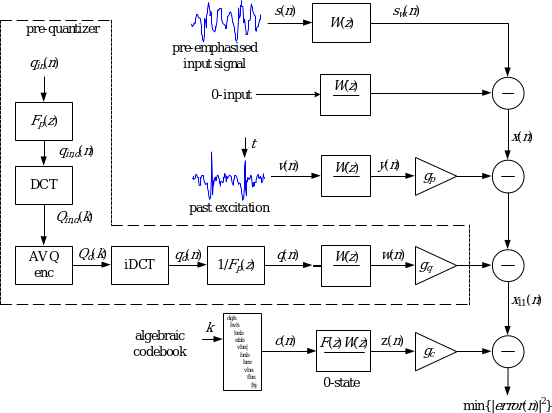Figure 21: Schematic diagram of the ACELP encoder using a combined algebraic codebook in GC mode at high bit-rates

At the encoder, the first stage, or pre-quantizer, operates as follows. In a given subframe (aligned to the subframe of the ACELP codebook in the second stage) the excitation residual after applying the adaptive codebook is computed as

(536)

where r(n) is the target vector in residual domain. Further, v(n) is the adaptive codevector and gp the adaptive codevector gain.

The excitation residual after applying the adaptive codebook is de-emphasized with a filter . A difference equation for such a de-emphasis filter is given by

(537)

where is the de-emphasized residual and coefficient controls the level of de-emphasis.

Further a DCT is applied to the de-emphasized excitation residual using a rectangular non-overlapping window. Depending on the bit rate, all blocks or only some blocks of DCT coefficients usually corresponding to lower frequencies are quantized using the AVQ encoder. The other (not quantized) DCT coefficients are set to 0 (not quantized). To obtain the excitation residual for the second (ACELP) stage of the combined algebraic codebook, the quantized DCT coefficients are inverse transformed, and then a pre-emphasis filter is applied to obtain the time-domain contribution from the pre-quantizer . The pre-emphasis filter has the inverse transfer function of the de-emphasis filter .

5.2.3.1.6.1 Quantization

The AVQ encoder produces quantized transform-domain DCT coefficients . The indices of the quantized and coded DCT coefficients from the AVQ encoder are transmitted as a pre-quantizer parameters to the decoder.

In every sub-frame, a bit-budget allocated to the AVQ is composed as a sum of a fixed bit-budget and a floating number of bits. Depending on the used AVQ sub-quantizers of the encoder, the AVQ usually does not consume all of the allocated bits, leaving a variable number of bits available in each sub-frame. These bits are floating bits employed in the following sub-frame. The floating number of bits is equal to 0 in the first sub-frame and the floating bits resulting from the AVQ in the last sub-frame in a given frame remain unused when coding WB signals or are re-used in coding of upper band (see subclause 5.2.6.3).

5.2.3.1.6.2 Computation of pre-quantizer gain

Once the pre-quantizer contribution is computed, the pre-quantizer gain is obtained as

(538)

where are the AVQ input frequency coefficients and the AVQ output (quantized) frequency coefficients where is the transform-domain coefficient index and being the number of DCT transform coefficients.

5.2.3.1.6.3 Quantization of pre-quantizer gain

The pre-quantizer gain is quantized as follows. First, the gain is normalized by the predicted innovation energy as follows: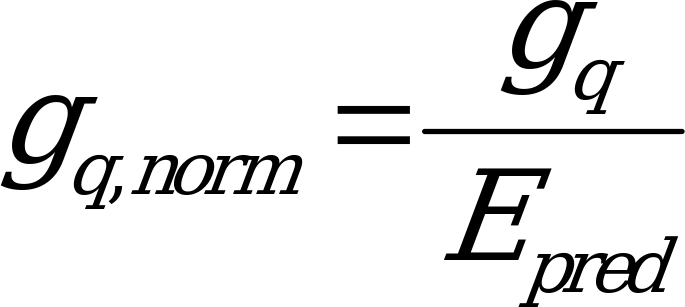(539)

where the predicted innovation energy is obtained as described in subclause 5.2.3.1.7.1.

Then the normalized gain is quantized by a scalar quantizer in a logarithmic domain and finally de-normalized resulting in a quantized pre-quantizer gain. Specifically 6-bit scalar quantizer is used whereby the quantization levels are uniformly distributed in the log domain. The index of the quantized pre-quantizer gain is transmitted as a pre-quantizer parameter to the decoder.

5.2.3.1.6.4 Refinement of target vector

The pre-quantizer contribution is used to refine the original target vector for adaptive codebook search as

, (537)

and to refine the adaptive codebook gain using equation (505) with used instead of . When the pre-quantizer is used, the computation of the target vector for algebraic codebook search is done using

(538)

where is the filtered pre-quantizer contribution, i.e. the zero-state response of the weighted synthesis filter to the pre-quantizer contribution , and is the refined adaptive codebook gain.

Similarly, the target vector in residual domain is updated for the algebraic codebook search (the second-stage of the combined algebraic codebook) as

. (539)

5.2.3.1.6.5 Combined algebraic codebook in GC mode

In the EVS codec, the combined algebraic codebook structure as from figure 21 is used at bit-rates of 32 kbps and 64 kbps. In both cases the algebraic codebook search uses 36-bit codebooks and the rest of the bit-budget is employed by the AVQ to quantize the pre-quantizer coefficients.

At 32 kbps, the available fixed bit-budget for the AVQ (116, 115, 115, 115, 155 bits for every of five subframes) is sometimes too low to properly encode all input signal frames. Consequently in GC mode at 32kbps, the DCT and iDCT stages of pre-quantizer computation are omitted when the input signal is not classified as a harmonic one. The classification is based on a harmonicity counter updated every frame in the pre-processing module. If in a given frame the harmonicity counter the frame is classified as non-harmonic and the AVQ is applied directly on the time-domain signal and similarly producing directly the time-domain signal in figure 21.

5.2.3.1.6.6 Combined algebraic codebook in TC mode

The combined algebraic codebook structure is used also in TC mode at 32kbps and 64kbps. In this mode the algebraic codebook from figure 21 is replaced by glottal shape codebook but the structure of the pre-quantizer remains the same as in the GC mode. In TC mode @32kbps, the DCT and iDCT stages of the pre-quantizer are always employed.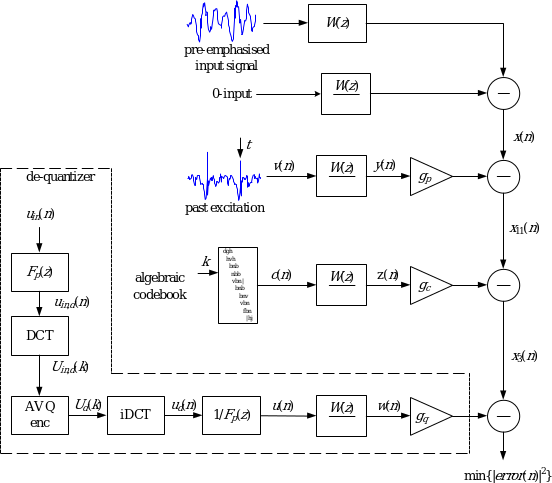Figure 22: Schematic diagram of the ACELP encoder using a combined algebraic codebook in IC mode at high bit-rates

5.2.3.1.6.7 Combined algebraic codebook in IC mode

Depending on the input signal characteristics, the ACELP encoder using a combined algebraic codebook from figure 21 is further adaptively changed. Specifically in coding of inactive speech segments, the order of the combined algebraic codebook stages is changed. I.e. the modified combined algebraic codebook combines a time-domain ACELP codebook in a first stage followed by a frequency-domain de-quantizer coding in a second stage as shown in figure 22. The first stage algebraic codebook employs very small codebooks, specifically 12 bits per subframe.

At the encoder, the de-quantizer in IC mode operates as follows. In a given subframe, the target signal after subtracting the scaled filtered adaptive excitation and the scaled filtered algebraic excitation is computed as

. (540)

The target signal in speech domain is filtered through the inverse of the weighted synthesis filter with zero states resulting in the target in residual domain .

Similarly to the combined algebraic codebook in GC mode, the signal is first de-emphasized with a filter to enhance the low frequencies. A DCT is applied to the de-emphasized signal using rectangular non-overlapping window. Usually all blocks of DCT coefficients are quantized using the AVQ encoder. The quantized DCT coefficients in some bands can be however set to zero.

The quantized DCT coefficients are further inverse transformed using iDCT, and then a pre-emphasis filter is applied to obtain the time-domain contribution from the frequency-domain quantizer where the pre-emphasis filter has the inverse transfer function of the de-emphasis filter .

5.2.3.1.6.8 Computation and quantization of de-quantizer gain

Once the de-quantizer contribution is computed, the de-quantizer gain is obtained as

(541)

where are the AVQ input transform-domain coefficients and are the AVQ output (quantized) transform-domain coefficients.

The de-quantizer gain is quantized using the normalization by the algebraic codebook gain . Specifically a 6-bit scalar quantizer is used whereby the quantization levels are uniformly distributed in the linear domain. The indice of the quantized de-quantizer gain is transmitted as a de-quantizer parameter to the decoder.

When coding the inactive signal segments the adaptive codebook excitation contribution is limited to avoid a strong periodicity in the synthesis. In practice a limiter is applied in the adaptive codebook search to constrain the adaptive codebook gain by .

5.2.3.1.6.9 AVQ quantization with split multi-rate lattice VQ

Prior to the AVQ quantization, the time domain or transform-domain 64 coefficients, here denoted as , are split into 8 consecutive sub‑bands of 8 coefficients each. The sub-bands are quantized with an 8-dimensional multi-rate algebraic vector quantizer. The AVQ codebooks are subsets of the Gosset lattice, referred to as the RE8 lattice.

5.2.3.1.6.9.1 Multi-rate AVQ with the Gosset Lattice RE8

5.2.3.1.6.9.1.1 Gosset Lattice RE8

The Gosset lattice RE8 is defined as the following union:

(542)

where is the 8-dimensional lattice composed of all points with integers components with the constraint that the sum of the 8 components is even. The lattice is simply the lattice scaled by 2. This implies that the sum of the components of a lattice point in is an integer multiple of 4. Therefore, the 8 components of a lattice point have the same parity (either all even or all odd) and their sum is a multiple of 4.

All points in the lattice RE8 lie on concentric spheres of radius , being the codebook number in sub-band . Each lattice point on a given sphere can be generated by permuting the coordinates of reference points called “leaders”. There are very few leaders on a sphere compared to the total number of lattice points which lie on the sphere.

5.2.3.1.6.9.1.2 Multi-rate codebooks in Gosset Lattice RE8

To form a vector codebook at a given rate, only lattice points inside a sphere in 8 dimensions of a given radius are taken. Codebooks of different bit rates can be constructed by including only spheres up to a given radius. Multi-rate codebooks are formed by taking subsets of lattice points inside spheres of different radii.

5.2.3.1.6.9.1.2.1 Base codebooks

First, base codebooks are designed. A base codebook contains all lattice points from a given set of spheres up to a number . Four base codebooks , noted , , , and , are used. There are 36 non-null absolute leaders plus the zero leader (the origin): Table 48 gives the list of these leaders and indicates to which codebook a leader belongs. , , , and are constructed with respectively 0, 8, 12, and 16 bits. Hence codebook requires bits to index any point in that codebook.

5.2.3.1.6.9.1.2.2 Voronoi extensions

From a base codebook (i.e. a codebook containing all lattice points from a given set of spheres up to a number ), an extended codebook can be generated by multiplying the elements of by a factor , and adding a second-stage codebook called the Voronoi extension. This construction is given by

(543)

where is the scaling factor, is a point in a base codebook and is a point in the Voronoi extension. The extension is computed in such a way that any point from equation (544) is also a lattice point in . The scaling factor is a power of 2 (), where is called the Voronoi extension order.

Such extended codebooks include lattice points that extend further out from the origin than the base codebook. When a given lattice point is not included in a base codebook (, , or ), the so-called Voronoi extension is applied, using the or base codebook part.

Giving the available bit-budget in particular layers, the maximum Voronoi extension order is . Therefore, for or , two extension orders are used: ().

When , there is no Voronoi extension, and only a base codebook is used.

5.2.3.1.6.9.1.2.3 Codebook rates

There are 8 codebooks: the first 4 are base codebooks without Voronoi extension and the last four with Voronoi extension. The codebook number is encoded as a unary code with "1" bits and a terminating "0". Table 49 gives for each of the 8 codebooks, its base codebook, its Voronoi extension order ( indicates that there is not Voronoi extension), and its unary code.

Table 50: Multi-rate codebooks in RE8 lattice

Codebook
number

Base Codebook

Voronoi extension order

Unary code for

0

Q0

0

0

2

Q2

0

10

3

Q3

0

110

4

Q4

0

1110

5

Q3

1

11110

6

Q4

1

111110

7

Q3

2

1111110

8

Q4

2

11111110

For the base codebook , (), there is only one point in the codebook and 1 bit is used to transmit the unary code corresponding to .

For the other three base codebooks () without Voronoi extension:

• bits are used to transmit the unary code corresponding to ,,
• bits are required to index a point in
• thus bits are used in total.

For codebooks with Voronoi extension ():

• bits are used to transmit the unary code corresponding to the base codebook number (respectively ) if is even (respectively odd) and the Voronoi extension order is 1 if , or 2 otherwise),
• 12 bits (respectively 16 bits) are required to index the point in the base codebook (respectively )
• bits are required to index the 8-dimensional point in the Voronoi extension of order
• thus, bits are used in total.

In the codebook number encoding, a simple bit overflow check is performed: in case when the last AVQ coded sub-band of the spectrum is quantized, and only bits are available for the quantization, the terminating "0" in the codebook number coding is not encoded. At the decoder, the same bit overflow check enables the right decoding of the codebook number in this sub-band.

5.2.3.1.6.9.2 Quantization with RE8 lattice

In lattice quantization, the operation of finding the nearest neighbour of the input spectrum among all codebook points is reduced to a few simple operations, involving rounding the components of spectrum and verifying a few constraints. Hence, no exhaustive search is carried out as in stochastic quantization, which uses stored tables. Once the best lattice codebook point is determined, further calculations are also necessary to compute the index that will be sent to the decoder. The larger the components of the input spectrum , the more bits will be required to encode the index of its nearest neighbour in the lattice codebook. Hence, to remain within a pre-defined bit-budget, a gain-shape approach has to be used, where the input spectrum is first scaled down by the AVQ gain, then each 8-dimensional block of spectrum coefficients is quantized in the lattice and finally scaled up again to produce the quantized spectrum.

5.2.3.1.6.9.2.1 AVQ gain estimation

Prior to the quantization (nearest neighbour search and indexation of the nearest neighbour), the input spectrum has to be scaled down to ensure that the total bit consumption will remain within the available bit-budget.

A first estimation of the total bit-budget without scaling (i.e. with an AVQ gain equals to 1) is performed:

(545)

where is a first estimate of the bit budget to encode the sub-band given by:

(546)

with being the energy (with a lower limit set to 2) of each sub-band :

(547)

This gain estimation is performed in an iterative procedure described below.

Let NB_BITS be the number of bits available for the quantization process and NB_SBANDS the number of 8-dimensional sub-bands to be quantized:

 Initialization:fac = 128,offset = 0,nbitsmax = 0.95 (NB_BITS – NB_SBANDS)for i = 1:10 offset = offset + fac if nbits ≤ nbitsmax, then offset = offset – fac fac = fac / 2

After the 10th iteration, the AVQ gain is equal to and is used to obtain the scaled spectrum :

(548)

5.2.3.1.6.9.2.2 Nearest neighbour search

The search of the nearest neighbour in the lattice RE8 is equivalent to searching for the nearest neighbour in the lattice and for the nearest neighbour in the lattice , and finally selecting among those two lattice points the closest to ) as its quantized version .

Based on the definition of , the following fast algorithm is used to search the nearest neighbour of an 8-dimensional sub-band among all lattice points in :

 Search of the nearest neighbour y1j in of :Compute .Round each component of to the nearest integer to generate .Compute .Calculate the sum of the 8 components of .if is not an integer multiple of 4, then modify its component as follows:whereSearch of the nearest neighbour in of :Compute where denotes an 8-dimensional vector with all ones.Round each component of to the nearest integer to generate .Compute .Calculate the sum S of the 8 components of .if S is not an integer multiple of 4 then modify its Ith component as follows:whereCompute .Select between and as the closest point in to :where and .

5.2.3.1.6.9.3 Indexation

The quantized scaled sub-band of ) is a point in a RE8 lattice codebook, an index for each has to be computed and later inserted into the bitstream.

This index is actually composed of three parts:

1. a codebook number ;
2. a vector index , which uniquely identifies a lattice vector in a base codebook ;
3. and if , an 8-dimensional Voronoi extension index that is used to extend the base codebook when the selected point in the lattice is not in a base codebook .

The calculation of an index for a given point in the lattice is performed as follows:

First, it is verified whether is in a base codebook by identifying its sphere and its leader:

• if is in a base codebook, the index used to encode is thus the codebook number plus the index of the lattice point in .

Otherwise, the parameters of the Voronoi extension (see equation (549)) have to determined: the scaling factor Mv, the base codebook ( or ), the point in this base codebook, and the point in the Voronoi extension. Then, the index used to encode is composed of the codebook number () plus the index of the lattice point in the base codebook ( or ), and the index of . in the Voronoi extension.

5.2.3.1.6.9.3.1 Indexing a codebook number

As explained in subclause 5.2.3.1.6.9.1.2.3 – Codebook rates, the codebook index is unary encoded with bits except for that is coded with one bit (see table 51).

5.2.3.1.6.9.3.2 Indexing of codevector in base codebook

The index Ij indicates the rank of codevector in j-th sub-band, i.e., the permutation to be applied to a specific leader to obtain . The index computation is done in several steps, as follows:

1) The input codevector is decomposed into a sign vector s0 and an absolute vector y0 following a two‑path procedure.

2) The sign vector is encoded, the associated index and the number of non-zero components in are obtained. More details are given in subsequent subclauses.

3) The absolute vector is encoded using a multi-level permutation-based index encoding method, and the associated index rank(y0) is obtained.

4) The absolute vector index and the sign index are added together in order to obtain the input vector rank: .

(550)

5) Finally, the offset is added to the rank. The index is obtained by

(551)

The indexing of codevector in base codebook is done in two steps. First the sign vector is encoded.

The number of bits required for encoding the sign vector elements is equal to the number of non‑zero elements in the codevector. "1" represents a negative sign and "0" a positive sign. As lattice quantization is used, the sum of all the elements in a codevector is an integer multiple of 4. If there is any change of sign in the non-zero element, the sum may not be a multiple of 4 anymore, in that case, the last element sign in the sign vector will be omitted. For example, the sign vector of the input vector (–1, –1, 1, 1, 1, 1, –1, –1) in leader 1 (see table 52) has seven bits and its value is 0x1100001.

In the second step the absolute vector and its position vector is encoded

The encoding method for the absolute vector works as follows. The absolute vector is first decomposed into MLmax levels. The highest-level vector is the original absolute vector. The value for is initialized to zero. Then:

1) First the intermediate absolute value vector of is obtained by removing the most frequent element as given in the decomposition order column of table 56 from the original absolute vector of. Sequentially the remaining elements are built into a new absolute vector for; it has a position order related to the level original absolute vector. All position values of the remainder elements are used to build a position vector of.

The relationship between the original absolute vector ofand the new absolute vector ofis that: the original absolute vector ofis the upper-level vector of the new absolute vector of, and the new absolute vector ofis the lower-level vector of the original absolute vector of.The relationship between any two neighbour level absolute vector is the same. The detail relationship is described as following: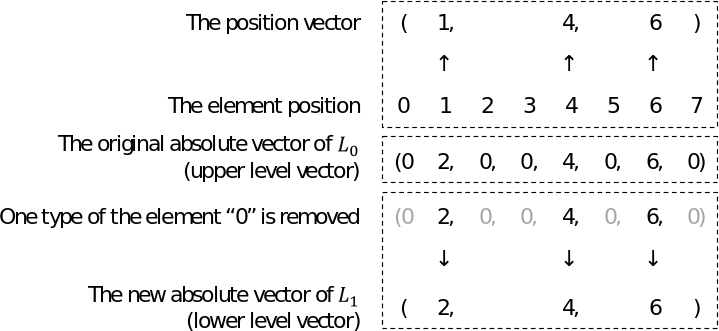Figure 23: Example processing of first level for.

2) Then the position vector of the new absolute vector of related to the original absolute vector of is indexed based on a permutation and combination function, the indexing result being called the middle index . For the new absolute vector in, the position vector indexing is computed as follows:

(552)

(553)

where is initialized to zero before the first step at the beginning of the procedure, is the dimension of the original absolute vector of, is the dimension of the new absolute vector of.

If there is more than one type of element in the new absolute vector, the new absolute vector, named the upper-level vector, will be encoded using the multi-level permutation-based index encoding method as following step:

3) Increment the n value. At level , , the intermediate absolute value vector is obtained by removing the most frequent element as given in the decomposition order column of table 53 from the upper-level vector. Sequentially the remaining elements are built into a new absolute vector for the current level; it has a position order related to the level absolute vector. All position values of the remainder elements are used to build a position vector.

4) The position vector of the current lower-level vector related to its upper-level vector is indexed based on a permutation and combination function, the indexing result being called the middle index . For the absolute vector in the current lower level, the position vector indexing is computed as follows:

(554)

(555)

The elements are the element values in the level position vector ranged from left to right according to their level, is the dimension of the upper-level absolute vector, is the dimension of the current-level absolute vector, represents the permutation and combination formula , , and . All the values forcan be stored in a simple table in order to avoid calculation of factorials. The level final-index, , is multiplied by the possible index value number, , in the current level and is added to the index, , in the current level, to obtain the final index, , of the current level.

5) Repeat steps 3 and 4 until there is only one type of element left in the current absolute vector. The for the lowest level is the rank of the absolute vector called . Table 54 is a sample extracted from the 36 leader table case. The leaders are indexed by . The decomposition order corresponds to the level order. The decomposition order column gives the order in which the element will be removed from the higher level. The last column gives the three class parameters, the first one is the number of sign bits, , the second one is the number of decomposition levels and equals the number of element types in the leader, , from the third one to the last one they represent the absolute vector dimension in each lower level except the highest level, (note that the dimension for the highest level is eight, but is not listed in table 55).

Table 56: List of leaders in base codebooks with their decomposition order and set parameter of multi-level permutation-based encoding

 Leader Decomposition order , , Q0 Q2 Q3 Q4 {0,0,0,0,0,0,0,0} X 0 {1,1,1,1,1,1,1,1} {1} {7,1} X X 1 {2,2,0,0,0,0,0,0} {0,2} {2,2,2} X X 2 {2,2,2,2,0,0,0,0} {0,2} {4,2,4} X 3 {3,1,1,1,1,1,1,1} {1,3} {7,2,1} X 4 {4,0,0,0,0,0,0,0} {0,4} {1,2,1} X X 5 {2,2,2,2,2,2,0,0} {2,0} {6,2,2} X 6 {3,3,1,1,1,1,1,1} {1,3} {7,2,2} X 7 {4,2,2,0,0,0,0,0} {0,2,4} {3,3,3,1} X 8 {2,2,2,2,2,2,2,2} {2} {8,1} X 9 {3,3,3,1,1,1,1,1} {1,3} {7,2,3} X 10 {4,2,2,2,2,0,0,0} {2,0,4} {5,3,4,1} X 11 {4,4,0,0,0,0,0,0} {0,4} {2,2,2} X 12 {5,1,1,1,1,1,1,1} {1,5} {7,2,1} X 13 {3,3,3,3,1,1,1,1} {1,3} {7,2,4} X 14 {4,2,2,2,2,2,2,0} {2,0,4} {7,3,2,1} X 15 {4,4,2,2,0,0,0,0} {0,2,4} {4,3,4,2} X 16 {5,3,1,1,1,1,1,1} {1,3,5} {7,3,2,1} X 17 { 6,2,0,0,0,0,0,0} {0,2,6} {2,3,2,1} X 18 { 4,4,4,0,0,0,0,0} {0,4} {3,2,3} X 19 { 6,2,2,2,0,0,0,0} {0,2,6} {4,3,4,1} X 20 { 6,4,2,0,0,0,0,0} {0,2,4,6} {3,4,3,2,1} X 21 { 7,1,1,1,1,1,1,1} {1,7} {7,2,1} X 22 { 8,0,0,0,0,0,0,0} {0,8} {1,2,1} X 23 {6,6,0,0,0,0,0,0} {0,6} {2,2,2} X 24 {8,2,2,0,0,0,0,0} {0,2,8} {3,3,3,1} X 25 {8,4,0,0,0,0,0,0} {0,4, 8} {2,3,2,1} X 26 {9,1,1,1,1,1,1,1} {1,9} {7,2,1} X 27 {10,2,0,0,0,0,0,0} {0,2,10} {2,3,2,1} X 28 {8,8,0,0,0,0,0,0} {0,8} {2,2,2} X 29 {10,6,0,0,0,0,0,0} {0,6,10} {2,3,2,1} X 30 {12,0,0,0,0,0,0,0} {0,12} {1,2,1} X 31 {12,4,0,0,0,0,0,0} {0,4,12} {2,3,2,1} X 32 {10,10,0,0,0,0,0,0} {0,10} {2,2,2} X 33 {14,2,0,0,0,0,0,0} {0,2,14} {2,3,2,1} X 34 {12,8,0,0,0,0,0,0} {0,8,12} {2,3,2,1} X 35 {16,0,0,0,0,0,0,0} {0,16} {1,2,1} X

The last value of the decomposition order for the leader is stored separately because this leader is the only one with 4 different values, the second dimension of the decomposition order being thus reduced from 4 to 3.

Figure 24 gives an encoding example for the leader .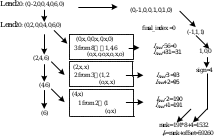Figure 32: Example processing for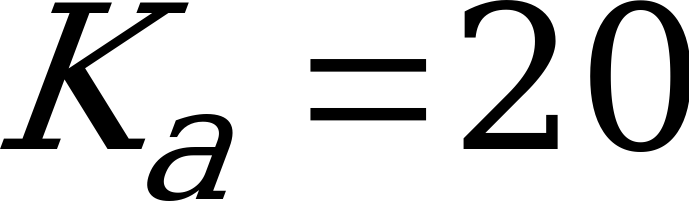.

For example, in case the input vector is {0,–2,0,0,4,0,6,0}, the absolute input vector will be {0,2,0,0,4,0,6,0}, its associated leader can be found for Ka. The set of decomposition order is {0,2,4,6}. For the highest level L0, element "0" is removed first from the absolute vector. The first level absolute vector is {2,4,6}, its position vector is {1,4,6}. The second element which will be removed is "2", the second level absolute vector is {4,6}, its position vector is {1,2}. The third element which will be removed is "4", the third level absolute vector is {6}, its position vector is {1}.

The absolute vectors that have only two different values, out of which the most frequent is zero, are treated separately in a less complex procedure combining the encoding of the position vector with the sign encoding. These vectors have generally higher probability of occurrence. Example of such vectors are those derived for instance from the leaders: (2,2,0,0,0,0,0,0), (2,2,2,2,0,0,0,0). For these vectors there is a single level for the creation of the index and the first level remaining elements are the non-null components which are the significant elements for the sign encoding. The determination of the remaining elements and the creation of the sign index can be done thus in a single loop.

5.2.3.1.6.9.4 Voronoi extension determination and indexing

If the nearest neighbour is not in the base codebook, then the Voronoi extension has to be determined through the following steps.

(a) Set the Voronoi extension order and the scaling factor .

(b) Compute the Voronoi index of the lattice point that depends on the extension order and the scaling factor . The Voronoi index is computed via component-wise modulo operations such that depends only on the relative position of in a scaled and translated Voronoi region:

(556)

where is the generator matrix. Hence, the Voronoi index is a vector of integers with each component in .

(c) Compute the Voronoi codevector from the Voronoi index . The Voronoi codevector is obtained as

(557)

where is the nearest neighbour of in infinite (see subclause 5.2.3.1.6.9.2.2for search details) and and are defined as

(558)

and

(559)

(d) Compute the difference vector . This difference vector always belongs to the scaled lattice . Compute , i.e. apply the inverse scaling to the difference vector . The codevector belongs to the lattice since belongs to lattice.

(e) Verify whether is in the base codebook (i.e. in or ).

If is not in C, increment the extension order by 1, multiply the scaling factor by 2, and go back to sub-step (b).

Otherwise, if is in C, then the Voronoi extension order has been found and the scaling factor is sufficiently large to encode the index of .

5.2.3.1.6.9.3 Insertion of AVQ parameters into the bitstream

The parameters of the AVQ in each sub-band j consist of the codebook number , the vector index in base codebook and the 8-dimensional Voronoi index . The codebook numbers are in the set of integers {0, 2, 3, 4, 5, 6, 7, 8} and the size of its unary code representation is bits with the exception of that requires 1 bit and a possible overflow in the last AVQ coded sub-band. The size of each index and is given by 4nj bits and bits, respectively.

The AVQ parameters , , , are written sequentially in groups corresponding to the same sub‑band into the corresponding bitstream as

. (560)

Note that if the lattice point in the block is in the base codebook , the Voronoi extension is not searched and consequently the index is not written into the bitstream in this group.

The actual bit-budget needed to encode AVQ parameters in current frame varies from sub-frame to sub-frame. The difference of bits between the allocated bits and actually spent bits are unused bits that can be employed in the subsequent sub-frame or high-rate higher band coding.

5.2.3.1.7 Gain quantization

5.2.3.1.7.1 Memory-less quantization of the gains

The adaptive codebook gain (pitch gain) and the algebraic codebook gain are quantized jointly in each subframe, using a 5-bit vector quantizer. While the adaptive codebook gain is quantized directly, the algebraic codebook gain is quantized indirectly, using a predicted energy of algebraic codevector. Note that, in this case, the prediction does not use any past information which limits the effect of frame-erasure propagation.

First, energy of residual signal in dB is calculated in each subframe as

(559)

where denotes the subframe and is the residual signal, defined in subclause 5.2.3.1.1. Then, average residual signal energy is calculated for the whole frame as

(560)

which is further modified by subtracting an estimate of the adaptive codebook contribution. That is

(561)

where and , are as defined in subclause 5.1.10.4, are the normalized correlations of the first and the second half-frames, respectively. The result of equation (562), , serves as a prediction of the algebraic codevector energy and is quantized with 3 bits once per frame. The quantized value of the predicted algebraic codevector energy is defined as

(563)

where is the n-bit codebook for the predicted algebraic codevector energy and is the index minimizing the criterion above. The bit allocation is bit-rate and mode dependant and is given in Table 57

Table 47: Predictor energy codebook bit allocation

 Rate (kbps) VC GC TC IC/UC 7.2 n.a. n.a. 4 n.a. 8 n.a. n.a. 4 n.a. 9.6 3 3 n.a. n.a. 13.2 5 4 4 n.a. 16.4 3 3 n.a. 3 24.4 3 3 n.a. 3 32 n.a. 5 5 5 64 n.a. 5 5 5

Now, let denote the algebraic codebook excitation energy in dB in a given subframe, which is given by

(564)

In the equation above, is the filtered algebraic codevector, found in subclause 5.2.3.1.5.

Using the predicted algebraic codevector energy and the calculated algebraic codebook excitation energy, we may estimate the algebraic codebook gain as

(565)

A correction factor between the true algebraic codebook gain, , and the estimated one, , is given by

(566)

The pitch gain,, and correction factor are jointly vector-quantized using a n-bit codebook, where n is dependent on the bit-rate and coding mode as shown in Table 48

Table 49: Gain codebook bit allocation per subframe

 Rate (kbps) VC GC UC/IC 7.2 7/6/6/6 6/6/6/6 n.a. 8 8/7/6/6 8/7/6/6 n.a. 9.6 5/5/5/5 5/5/5/5 n.a. 13.2 6/6/6/6 6/6/6/6 n.a. 16.4 7/7/7/7/7 7/7/7/7/7 6/6/6/6/6 24.4 7/7/7/7/7 7/7/7/7/7 6/6/6/6/6 32 6/6/6/6/6 6/6/6/6/6 6/6/6/6/6 64 12/12/12/12/12 12/12/12/12/12 6/6/6/6/6

The gain codebook search is performed by minimizing a mean-squared weighted error between the original and the reconstructed signal, which is given by

(567)

where is the target vector, is the filtered adaptive codevector, and is the filtered algebraic codevector. The quantized value of the pitch gain is denoted asand the quantized value of the algebraic codebook gain is denoted as , where is the quantized value of the factor .

Furthermore, if pitch gain clipping is detected (as described in subclause 5.2.3.1.4.2), the last 13 entries in the codebook are skipped in the quantization procedure since the pitch gain in these entries is higher than 1.

5.2.3.1.7.2 Memory-less joint gain coding at lowest bit-rates

For the lowest bitrates of 7.2 and 8.0 kbps, slightly different memory-less joint gain coding scheme is used. This is due to the fact that there are not enough bits to cover the dynamic range of the target vector for algebraic search.

In the first subframe of the current frame, the estimated (predicted) gain of algebraic codebook is given by

(568)

where CT is a signal classification parameter (the coding mode), selected for the current frame in the pre-processing part, and is the energy of the filtered algebraic codevector, calculated in equation (569). The inner term inside the logarithm corresponds to the gain of innovation vector. The constants a0 and a1 are found by means of MSE minimization on a large signal database. The only parameter in the equation above is the coding mode CT which is constant for all subframes of the current frame. The superscript  denotes the first subframe of the current frame. The estimation process for the first subframe is schematically depicted in the figure below.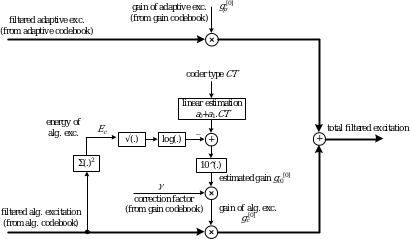Figure 33: Schematic description of the calculation process of algebraic gain in the first subframe

All subframes following the first subframes use slightly different estimation scheme. The difference is in the fact that in these subframes, the quantized gains of both the adaptive and the algebraic codebook from previous subframe(s) are used as auxiliary estimation parameters to increase the efficiency. The estimated value of the algebraic codebook gain in kth subframe, k>0 is given by

(570)

where k=1,2,3. Note, that the terms in the first and in the second sum of the exponent, there are quantized gains of algebraic and adaptive excitation of previous subframes, respectively. Note that the term including the gain of innovation vector is not subtracted. The reason is in the use of the quantized values of past algebraic codebook gains which are already close enough to the optimal gain and thus it is not necessary to subtract this gain again. The estimation constants b0,…,b2k+1 are found again through MSE minimization on a large signal database. The gain estimation process for the second and the following subframes is schematically depicted in the figure below.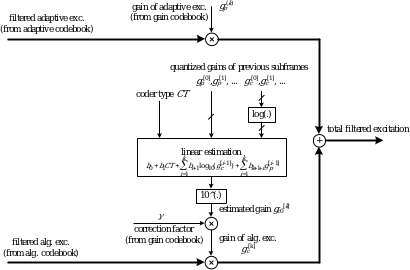Figure 34: Schematic description of the calculation process of algebraic gain in the following subframes

The gain quantization is done both at the encoder and at the decoder by searching the gain codebook and evaluating the MMSE between the target signal and the filtered adaptive codeword. In each subframe, the codebook is searched completely, i.e. for q=0,..,Q-1 where Q is the number of codebook entries. It is possible to limit the searching range in case ĝp is mandated to lie below certain threshold. To allow reducing the search range, the codebook entries are sorted in ascending order according to the value of ĝp.

The gain quantization is performed by calculating the following MMSE criterion for each codebook entry

(571)

where the constants c0, c1, c2, c3, c4 and c5 are calculated as

(572)

in which x(i) is the target signal, y(i) is the filtered adaptive excitation signal and z(i) is the filtered algebraic excitation signal. The codevector leading to the lowest energy is chosen as the winning codevector and its entries correspond to the quantized values of gp and .

Before the gain quantization process it is assumed that both the filtered adaptive and innovation codewords are already known. The gain quantization at the encoder is performed by searching the designed gain codebook in the MMSE sense. Each entry in the gain codebook consists of two values: the quantized gain of the adaptive part and the correction factor for the algebraic part of the excitation. The estimation of the algebraic gain excitation is done beforehand and the resulting gc0 is used to multiply the correction factor selected from the codebook. In each subframe the gain codebook is searched completely, i.e. for q=0,..,Q-1. It is possible to limit the search range if the quantized gain of the adaptive part of the excitation is mandated to be below certain threshold. To allow for reducing the search range, the codebook entries are sorted in ascending order according to the value of gp. The gain quantization process is schematically depicted in the figure below.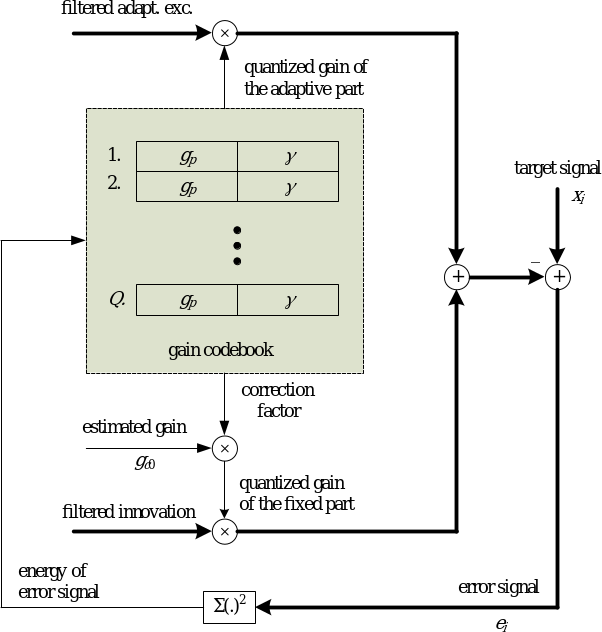Figure 35: Schematic diagram of the gain quantization process in the encoder

The gain quantization is performed by minimizing the energy of the error signal e(i) The error energy is given by

(573)

By replacing by we obtain

(574)

The constants c0, c1, c2, c3, c4 and c5 and the estimated gain are computed before the search of the gain codebook. The error energy E is calculated for each codebook entry. The codevector [;] leading to the lowest error energy is selected as the winning codevector and its entries correspond to the quantized values of gp and . The quantized value of the fixed codebook gain is then calculated as

(575)

In the decoder, the received index is used to retrieve the values of the quantized gain of the adaptive excitation and the quantized correction factor of the estimated gain of the algebraic excitation. The estimated gain for the algebraic part of the excitation is done in the same way as in the encoder.

5.2.3.1.7.3 Scalar gain coding at highest bit-rates

At the bit-rate of 64kbps and at the last subframe of TC7 and TC165 (see later in subclause 5.2.3.2.2), the adaptive codebook gain (pitch gain) and the algebraic codebook gain are quantized using a scalar quantizers. The adaptive codebook gain is quantized using a uniform scalar quantizer according to MMSE criterion in the range between [0; 1.22]. In contrast the quantized algebraic codebook gain is obtained as a product of a correction factor and the estimated algebraic codebook gain , see equation 566, where the correction factor is quantized in log domain in the range between [0.02; 5.0].

At 64 kbps, both the adaptive codebook gain and the algebraic codebook gain are quantized by means of 6 bits each. In the last subframe of TC configurations TC7 and TC165, they are quantized by means of 6-8 bits depending on the bit-rate.

5.2.3.1.8 Update of filter memories

An update of the states of the synthesis and weighting filters is needed in order to compute the target signal in the next subframe.

After the two gains have been quantized, the excitation signal,, in the present subframe is found by

(576)

where andare the quantized adaptive and algebraic codebook gains, respectively, is the adaptive codevector (interpolated, low-pass filtered past excitation), and is the algebraic codevector (including pre-filtering). The states of the filters can be updated by filtering the signal (difference between the residual signal and the excitation signal) through the filters and and saving the states of the filters. This would require 3 stages of filtering. A simpler approach, which requires only one filtering, is as follows. The local synthesis signal (without excitation post-processing) from layer is computed by filtering the excitation signal through. The output of the filter due to the input is equivalent to. So, the states of the synthesis filterare given by .

The updating of the states of the filtercan be done by filtering the error signal through this filter to find the perceptually weighted error . However, the signal can be equivalently found by

(577)

where is the adaptive codebook search target signal, is the filtered adaptive codebook vector, and is the filtered algebraic codebook vector. Since the signals , , and are available, the states of the weighting filter are updated by computing as in equation 578 for . This saves two stages of filtering.

5.2.3.2 Excitation coding in TC mode

The principle of excitation coding in TC mode is shown on a schematic diagram in Figure 36. The individual blocks and operations are described in detail in the following clauses.

5.2.3.2.1 Glottal pulse codebook search

The TC mode improves the robustness of the codec to frame erasures. It also encodes frames with an outdated past excitation buffer, e.g. after switching from HQ core frame.

The TC mode in the current frame is selected based on the classification algorithm described in subclause 5.1.13. The increased robustness, or the excitation building when the past excitation is outdated, is achieved by replacing the adaptive codebook (inter-frame long-term prediction) with a codebook of glottal impulse shapes (glottal-shape codebook) , which is independent from past excitation. The glottal-shape codebook consists of quantized normalized shapes of the truncated glottal impulses placed at specific positions. The codebook search consists of both the selection of the best shape and the best position.

To select the best codevector, the mean-squared error between the target signal, (the same target signal as used for the adaptive codebook search described in subclause 5.2.3.1.2), and the contribution signal, , is minimized for all candidate glottal-shape codevectors. The glottal-shape codebook search has been designed in a similar way as the algebraic codebook search, described in subclause 5.2.3.1.5.9. In this approach, each glottal shape is represented as an impulse response of a shaping filter. This impulse response can be integrated in the impulse response of the weighted synthesis filter prior to the search of the optimum impulse position. The searched codevectors can then be represented by vectors containing only one non-zero element corresponding to candidate impulse positions, and they can be searched very efficiently. Once selected, the position codevector is convolved with the impulse response of the shaping filter. This procedure needs to be repeated for all the candidate shapes and the best shape-position combination will form the excitation signal.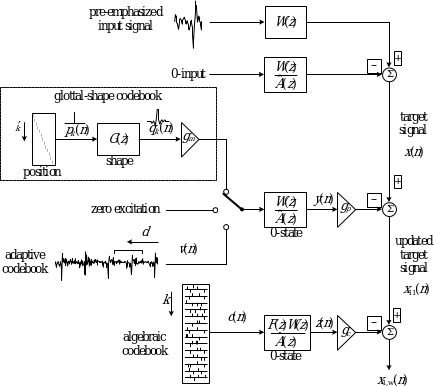Figure 37: Schematic diagram of the excitation coding in TC mode

In the following, all vectors are supposed to be column vectors. Let be a position codevector with one non-zero element at a position, and the corresponding glottal-shape codevector with index representing the centre of the glottal shape. Index is chosen from the range [0, 63], where 64 is the subframe length. Note that, due to the non-causal nature of the shaping filter, its impulse response is truncated for positions in the beginning and at the end of the subframe. The glottal shape codevector can be expressed in a matrix form as , where is a Toeplitz matrix representing the glottal impulse shape. Similarly to the algebraic codebook search, we can write

(579)

where is a lower triangular Toeplitz convolution matrix of the weighted synthesis filter. The rows of a convolution matrix correspond to the filtered shifted version of the glottal impulse shape or its truncated representation.

Because of the fact that the position codevector has only one non-zero sample, the computation of the criterion (580) is very simple and can be expressed as

(581)

As it can be seen from criterion (582), only the diagonal of the correlation matrix from criterion (583) needs to be computed.

The codebook consists of 8 prototype glottal impulse shapes of length samples placed at all subframe positions. Note that, since is shorter than the subframe length, the remaining samples in the subframe are set to zero.

In general, the coding efficiency of the glottal-shape codebook is lower than the efficiency of the long-term prediction, and more bits are generally needed to assure good synthesized speech quality.

However, the glottal-shape codebook does not need to be used in all subframes. First, there is no reason to use this codebook in subframes that do not contain any significant glottal impulse in the residual signal. Second, the glottal-shape codebook search is important only in the first pitch period in a frame. The following pitch periods can be encoded using the more efficient standard adaptive codebook search as it does not use the excitation of the past frame anymore. To satisfy the constant bit-rate requirement, the glottal-shape codebook is used in the EVS codec only in one of the four subframes in a frame. This leads to a highly structured coding mode where the bit allocation is dependent on the position of the first glottal impulse and the pitch period. The subframe where the glottal-shape codebook is used is chosen as the subframe with the maximum sample in the residual signal in the range , where is the open-loop pitch period estimated over the first half of the frame. The other subframes are processed as described in subclause 5.2.3.2.2.

Criterion (584) is typically used in the algebraic codebook search by pre-computing the backward filtered target vector and the correlation matrix . Given the non-causal nature of the filter , the matrix is not triangular and Toeplitz anymore, and this approach cannot be efficiently applied for the first positions in the glottal-shape codebook search.

Let be the th row of the matrix , where is computed in two steps to minimize the computational complexity. In the first step, the first rows of this matrix are calculated that correspond to the positions from the range . In the second step, the criterion (585) is used in a similar way as in the algebraic codebook search for the remaining part of (the last rows of the matrix ).

In the first step, the convolution between the glottal-shape codebook entry for position and the impulse response is first computed using

(586)

where we take advantage of the fact that the filter has only non-zero coefficients.

Next, the convolution between the glottal-shape codebook entry for the position and the impulse response is computed, reusing the values of . For the following rows, the recursion is reused, resulting in

(587)

The recursion (588) is repeated for all.

Now, the criterion (589) can be computed for all positions from the range in the form

(590)

In the second step, we take advantage of the fact that rows of the matrix are built using the coefficients of the convolution that are already computed as described by recursion (591) for . That is, each row corresponds to the previous row shifted to the right by 1 with a zero added at the beginning

(592)

and this is repeated for from the range .

Next, the target vector and the diagonal of the matrix need to be computed. First, we evaluate the numerator and the denominator of the criterion (593) for the last position

(594)

and

(595)

For the remaining positions, the numerator is computed using equation (596), but with the summation index changed. In the computation of the denominator, some of the previously computed values can be reused. For example, for the position , the denominator of criterion (597) is computed using

(598)

Similarly, we can continue to compute the numerator and the denominator of criterion (599) for all positions .

The search continues using the previously described procedure for all other glottal impulse shapes and the codevector corresponding to the best combination of glottal-shape and position is selected. To maintain the complexity low, the computation described above is further reduced by limiting the position search to ±4 samples around the maximum absolute value of the residual signal.

The last parameter to be determined is the gain of the glottal-shape codebook excitation. The gain is quantized in two steps. First, a roughly quantized gain of the glottal-shape codevector, , is found. Then, after both the first-stage contribution (glottal-shape codevector) and the second-stage contribution (algebraic codevector) of the excitation signal are found, the gain of the first-stage contribution signal is jointly quantized with the second-stage contribution gain, . This is done using the memory-less gain vector quantization, as described in subclause 5.2.3.1.7.1. The found glottal shape codevector is thus the position codevector filtered through the shaping filter that represents the best found glottal shape. When scaling the glottal-shape codevector with the signed quantized gain, we finally obtain the first stage excitation codevector, .

The glottal-shape gain is quantized using a quantization table as follows. First, an unquantized gain in the current glottal-shape subframe is found as

(600)

where is the subframe length, is the target signal and is the glottal-shape codevector filtered through the weighted synthesis filter. Further, the sign of the glottal-shape gain is set to 0 if and 1 otherwise, and written to the bitstream. Finally, the glottal-shape gain quantization index is found as the maximum value of that satisfies , where is the glottal-shape gain quantization table of dimension 8. The signed quantized glottal-shape gainis thus found as and its value is quantized using 4 bits (1 bit for sign, 3 bits for the value).

It should be noted that the closed-loop pitch period, , does not need to be transmitted anymore in a subframe which uses the glottal-shape codebook search with the exception of subframes containing more than one glottal impulse, i.e., when. There are situations where the pitch period of the input signal is shorter than the subframe length and, in this case, we have to transmit its value. Given the pitch period length limitations and the subframe length, a subframe cannot contain more than two impulses. In the situation that the glottal-shape codevector contains two impulses, an adaptive codebook search is used in a part of the subframe. The first samples of the glottal-shape codevector are built using the glottal-shape codebook search and then the other samples in the subframe are built using the adaptive search as shown in Figure 38.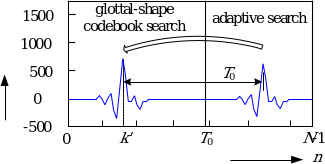Figure 39: Glottal-shape codevector with two impulses construction

The described procedure is used even if the second glottal impulse appears in one of the first positions of the next subframe. In this situation, only a few samples (less than ) of the glottal shape are used at the end of the current subframe. This approach has a limitation because the pitch period value transmitted in these situations is limited to , if it is bigger, it is not transmitted.

In order to enhance the coding performance, a low-pass filter is applied to the first stage excitation signal . In all subframes after the glottal-shape codebook subframe, the low-pass filtered first stage excitation is found as described in subclause 5.2.3.1.4.2.

5.2.3.2.2 TC frame configurations

5.2.3.2.2.1 TC frame configurations at 12.8 kHz internal sampling

At bit-rates with 12.8 kHz internal sampling rate the glottal-shape codebook is used in one out of four subframes. The other subframes in a TC frame (not encoded with the use of the glottal-shape codebook) are processed as follows. If the subframe with glottal-shape codebook search is not the first subframe in the frame, the excitation signal in preceding subframes is encoded using the algebraic CELP codebook only, this means that the first stage contribution signal is zero. If the glottal-shape codebook subframe is not the last subframe in the frame, the following subframes are processed by the standard CELP coding (i.e., using the adaptive and the algebraic codebook search). Thus, the first stage excitation signal is the scaled glottal-shape codevector, the adaptive codevector or the zero codevector.

In order to further increase encoding efficiency and to optimize bit allocation, different processing is used in particular subframes of a TC frame dependent on the pitch period. When the first subframe is chosen as a TC subframe, the subframe with the 2nd glottal impulse in the LP residual signal is determined. This determination is based on the pitch period value and the following four situations then can appear. In the first situation, the 2nd glottal impulse is in the 1st subframe, and the 2nd, 3rd and 4th subframes are processed using the standard CELP coding (adaptive and algebraic codebook search). In the second situation, the 2nd glottal impulse is in the 2nd subframe, and the 2nd, 3rd and 4th subframes are processed using the standard CELP coding again. In the third case, the 2nd glottal impulse is in the 3rd subframe. The 2nd subframe is processed using algebraic codebook search only as there is no glottal impulse in the 2nd subframe of the LP residual signal to be searched for using the adaptive codebook. The 3rd and 4th subframes are processed using the standard CELP coding. In the last (fourth) case, the 2nd glottal impulse is in the 4th subframe (or in the next frame), the 2nd and 3rd subframes are processed using the algebraic codebook search only, and the 4th subframe is processed using the standard CELP coding. Table 50 shows all possible coding configurations in the EVS codec at 12.8 kHz internal sampling rate.

The TC configuration is transmitted in the bit-stream using a Huffman-style coding and its bit sequence is show in the Table 50 in the column bitstream.

Table 50: TC configurations used in the EVS codec at 12.8 kHz internal sampling rate

 Coding configuration Bitstream Positions of the first (and the second, if relevant) glottal impulse(s) in the frame Type of codebook used (GS = glottal-shape,Ada = adaptive, Alg = algebraic) 1st subfr. 2nd subfr. 3rd subfr. 4th subfr. TC1 1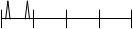GS + Alg Ada + Alg Ada + Alg Ada + Alg TC2 0101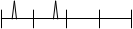GS + Alg Ada + Alg Ada + Alg Ada + Alg TC3 0100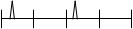GS + Alg Alg Ada + Alg Ada + Alg TC4 011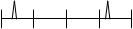GS + Alg Alg Alg Ada + Alg TC5 001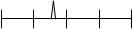Alg GS + Alg Ada + Alg Ada + Alg TC6 0001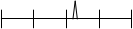Alg Alg GS + Alg Ada + Alg TC7 0000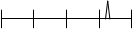Alg Alg Alg GS + Alg

5.2.3.2.2.2 TC frame configurations at 16 kHz internal sampling

At bit-rates with 16 kHz internal sampling rate the glottal-shape codebook is used in one out of five subframes. If the subframe with glottal-shape codebook search is not the first subframe in the frame, the excitation signal in preceding subframes is encoded using the algebraic CELP codebook only. If the glottal-shape codebook subframe is not the last subframe in the frame, the following subframes are processed by the standard CELP coding.

As the bit-rates with 16 kHz internal sampling rate are with high bit-budget, the number of TC configurations is reduced compared to the 12.8 kHz internal sampling rate. Table 51 shows all possible coding configurations in the EVS codec at 16 kHz internal sampling rate

Table 51: TC configurations used in the EVS codec at 16 kHz internal sampling rate

 Coding configuration Bitstream Positions of the first glottal impulse in the frame Type of codebook used(GS = glottal-shape,Ada = adaptive, Alg = algebraic) 1st subfr. 2nd subfr. 3rd subfr. 4th subfr. 5th subfr. TC161 00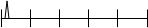GS + Alg Ada + Alg Ada + Alg Ada + Alg Ada + Alg TC162 01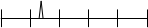Alg GS + Alg Ada + Alg Ada + Alg Ada + Alg TC163 10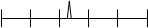Alg Alg GS + Alg Ada + Alg Ada + Alg TC164 110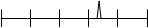Alg Alg Alg GS + Alg Ada + Alg TC165 111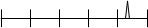Alg Alg Alg Alg GS + Alg

5.2.3.2.2.3 Pitch period and gain coding in the TC mode

When using the TC, it is not necessary to transmit the pitch period for certain subframes. Further, it is not necessary to transmit both pitch gain, , and the algebraic codebook gain, , for subframes where there is no important glottal impulse, and only the algebraic codebook contribution is computed (the first stage excitation is the zero vector).

In subframes, where the glottal-shape, or adaptive, search is used, the first stage excitation gain (pitch gain), , and the second stage excitation gain (algebraic gain), , are quantized at bit-rates ≤ 32 kbps using the memory-less vector gain quantization described in subclause 5.2.3.1.7.1. At 64 kbps bit-rate, gains are scalar quantized as described in subclause 5.2.3.1.7.3. In glottal-shape subframes, the first stage gain, , is found in the same manner as described in subclause 5.2.3.1.4.2.

When only an algebraic gain is quantized in the current frame (the first stage excitation is the zero vector), the following scalar quantization process is used. First, an optimal algebraic gain in the current subframe is found as

(601)

where is the subframe length, is the target signal and is the algebraic codevector filtered through the weighted synthesis filterwith the pre-filter . The predictive algebraic energy calculated once per frame is employed as described in subclause 5.2.3.1.7. Further the algebraic codebook gain, , and the correction factor, , are given by equations (602) and (603), respectively. Finally, the correction factor quantization index, , is found as the maximum value of that satisfies

(604)

where is the algebraic gain quantization table of dimension 8. The correction factor is quantized with 3 bits using the quantization tableand the quantized algebraic gain is obtained by

(605)

The following is a list of all TC configurations corresponding to Table 52 and Table 53.

Configuration TC1

In this configuration, two first glottal impulses appear in the first subframe that is processed using the glottal-shape codebook search. This means that the pitch period value in the 1st subframe can have the maximum value less than the subframe length, i.e., . Here, is the closed-loop pitch period and the subframe length. With the ½ sample resolution it can be coded with 6 bits. The pitch periods in the next subframes are found using – depending on the bit-rate – a 5- or 6-bit delta search with a fractional resolution.

Configuration TC2

When configuration TC2 is used, the first subframe is processed using the glottal-shape codebook search. The pitch period is not needed and all following subframes are processed using the adaptive codebook search. Because we know that the 2nd subframe contains the second glottal impulse, the pitch period maximum value holds . This maximum value can be further reduced thanks to the knowledge of the glottal impulse position value . The pitch period value in the 2nd subframe is then coded using 7 bits with a fractional resolution in the whole range of . In the 3rd and 4th subframes, a delta search using 6 bits is used with a fractional resolution.

Configuration TC3

When configuration TC3 is used, the first subframe is processed using the glottal-shape codebook search with no use of the pitch value again. But because the 2nd subframe of the LP residual signal contains no glottal impulse and the adaptive search is useless, the first stage contribution signal is replaced by zeros in the 2nd subframe. The adaptive codebook parameters ( and ) are not transmitted in the 2nd subframe. The first stage contribution signal in the 3rd subframe is constructed using the adaptive codebook search with the pitch period maximum value and the minimum value , thus only a 7-bit coding of the pitch value with fractional resolution in all range is needed. The 4th subframe is processed using the adaptive search with – depending on the bit-rate – a 5- or 6-bit delta search coding of the pitch period value.

In the 2nd subframe, only the algebraic codebook gain, gc, is transmitted. Consequently, only 3 bits are needed for gain quantization in this subframe as described at the beginning of this subclause.

Configuration TC4

When configuration TC4 is used, the first subframe is processed using the glottal-shape codebook search. Again, the pitch period does not need to be transmitted. But because the LP residual signal contains no glottal impulse in the 2nd and also in the 3rd subframe, the adaptive search is useless for both these subframes. Again, the first stage excitation signal in these subframes is replaced by zeros. The pitch period value is transmitted only in the 4th subframe by means of 7 bits and its minimum value is .The maximum value of the pitch period is limited by the value only. It does not matter if the second glottal impulse will appear in the 4th subframe or not (the second glottal impulse can be present in the next frame if ).

Note that the absolute value of the pitch period is necessary at the decoder for the frame concealment; therefore, it is transmitted also in the situation when the second glottal impulse appears in the next frame. When a frame preceding the TC frame is missing, the correct knowledge of the pitch period value from the frames and helps to reconstruct the missing part of the synthesis signal in the frame successfully.

The algebraic codebook gain,, is quantized with 3 bits in the 2nd subframe and 3rd subframe.

Configuration TC5

When the first glottal impulse appears in the 2nd subframe, the pitch period is transmitted only for the 3rd and 4th subframe. 3rd subframe, the pitch value is coded using 9-bit absolute search while in the 4th subframe using – depending on the bit-rate – 5- or 6- bits delta search. In this case, only algebraic codebook parameters are transmitted in the 1st subframe (with the algebraic codebook gain, , quantized with 3 bits).

Configuration TC6

When the first glottal impulse appears in the 3rd subframe, the pitch period does not need to be transmitted for the TC technique. In this case, only algebraic codebook parameters are transmitted in the 1st and 2nd subframe with the algebraic codebook gain,, quantized with 3 bits in both subframes. Nevertheless, the pitch period is transmitted in the 4th subframe by means of 9 bit absolute search coding for the reason of better frame erasure concealment in the frame after the TC frame. Also, the pitch period is transmitted for the 3rd subframe by means of 5 bit absolute search coding although it is not usually necessary.

Configuration TC7

When the first glottal impulse appears in the 4th subframe, the pitch period value information is not usually used in this subframe. However, its value is necessary for the frame concealment at the decoder (this value is used for the missing frame reconstruction when the frame preceding or following the TC frame is missing) or in case of strong onsets at the frame-end and very short pitch period. Thus, the pitch value is transmitted only in the 4th subframe by means of 9-bit absolute search coding and only algebraic codebook parameters are transmitted in the first three subframes (the gain pitch, , is not essential). The algebraic codebook gain, , is quantized with 3 bits in the 1st, 2nd and 3rd subframes. The scalar gain quantization is employed only at the 4th subframe in this configuration to encode the gain pitch and the algebraic codebook gain.

Configuration TC161

In this configuration, one or two first glottal impulses appear in the first subframe that is processed using the glottal-shape codebook search. This means that the pitch period value in the 1st subframe can have the maximum value less than the subframe length , i.e., and it is coded with 6 bits. Then the pitch period in the 2nd subframe is found using 8-bit absolute search on the interval . Finally the pitch period in the 3rd subframe is coded using 10-bit absolute search and in the 4th and 5th subframe using 6-bit delta search.

The gain pitch and the algebraic codebook gain are coded in all subframes using 6-bit VQ at 32 kbps resp. 12-bit SQ at 64 kbps.

Configuration TC162

The first glottal impulse appears in the 2nd subframe and the pitch period is transmitted for the 3rd, 4th and 5th subframe. In the 3rd subframe, the pitch value is coded using 10-bit absolute search while in the 4th and 5th subframe using 6- bits delta search. The pitch period is transmitted also in the 2nd subframe by means of 6 bits and serves in case when two first glottal impulses appears in the second subframe.

The gain pitch and the algebraic codebook gain are coded in the 2nd, 3rd, 4th and 5th subframe using 6-bit VQ at 32 kbps resp. 12-bit SQ at 64 kbps. The algebraic codebook gain,, is quantized in the 1st subframe with 3 bits at 32 kbps resp. 6 bits at 64 kbps

Configuration TC163

The first glottal impulse appears in the 3rd subframe. In this case, only algebraic codebook parameters are transmitted in the 1st and 2nd subframe with the algebraic codebook gain,, quantized in both subframes with 3 bits at 32 kbps resp. 6 bits at 64 kbps. Then the pitch period is coded by means of 10-bit absolute search in the 3rd subframe and by means of 6-bit delta search in the 4th and 5th subframe.

The gain pitch and the algebraic codebook gain are coded in the 3rd, 4th and 5th subframe using 6-bit VQ at 32 kbps resp. 12-bit SQ at 64 kbps.

Configuration TC164

The first glottal impulse appears in the 4th subframe. In this case, only algebraic codebook parameters are transmitted in the 1st, 2nd and 3rd subframe with the algebraic codebook gain,, quantized in all these subframes with 3 bits at 32kbps resp. 6 bits at 64kbps. Then the pitch period is coded by means of 10-bit absolute search in the 4th subframe and by means of 6-bit delta search in the 5th subframe.

The gain pitch and the algebraic codebook gain are coded in the 4th and 5th subframe using 6-bit VQ at 32 kbps resp. 12-bit SQ at 64 kbps.

Configuration TC165

When the first glottal impulse appears in the 5th subframe, the pitch period value information is not usually used in this subframe. However, its value is necessary for the frame concealment at the decoder (this value is used for the missing frame reconstruction when the frame preceding or following the TC frame is missing) or in case of strong onsets at the frame-end and very short pitch period. Thus, the pitch value is transmitted only in the 5th subframe by means of 10-bit absolute search coding and only algebraic codebook parameters are transmitted in the first four subframes. The algebraic codebook gain, , is quantized in the 1st, 2nd and 3rd subframes with 3 bits at 32kbps and 6 bits at 64 kbps. The gain pitch and the algebraic codebook gain are coded only in 5th subframe using a scalar gain quantizer.

5.2.3.2.2.4 Update of filter memories

In TC mode, the memories of the synthesis and weighting filter are updated as described in subclause 5.2.3.1.8. Note that signals in equation (606) are the first stage excitation signal (i.e., the glottal-shape codevector, the low-pass filtered adaptive codevector, or the zero codevector) and the algebraic codevector (including pre-filtering).

5.2.3.3 Excitation coding in UC mode at low rates

The principle of excitation coding in UC mode is shown in a schematic diagram in Figure 40. The individual operations are described in detail in the following clauses.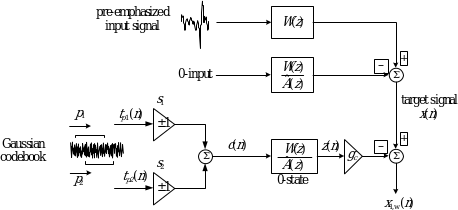Figure 38: Schematic diagram of the excitation coding in UC mode

5.2.3.3.1 Structure of the Gaussian codebook

In UC mode, a Gaussian codebook is used for representing the excitation. To simplify the search and reduce the codebook memory requirement, an efficient structure is used whereby the excitation codevector is derived by the addition of 2 signed vectors taken from a table containing 64 Gaussian vectors of dimension 64 (the subframe size). Let denote the th 64-dimensional Gaussian vector in the table. Then, a codevector is constructed by

(607)

where and are the signs, equal to –1 or 1, and and are the indices of the Gaussian vectors from the table. In order to reduce the table memory, a shift-by-2 table is used, thus only 64 + 63 × 2 = 190 values are needed to represent the 64 vectors of dimension 64.

To encode the codebook index, one has to encode 2 signs, and , and two indices, and . The values of and are in the range , so they need 6 bits each, and the signs need 1 bit each. However, 1 bit can be saved since the order of the vectors and is not important. For example, choosing as the first vector and as the second vector is equivalent to choosing as the first vector and as the second vector. Thus, similar to the case of encoding two pulses in a track, only one bit is needed for both signs. The ordering of the vector indices is such that the other sign information can be easily deduced. This gives a total of 13 bits. To better explain this procedure, let us assume that the two vectors have the indices and with sign indices and , respectively (if the sign is positive and if the sign is negative). The codevector index is given by

(608)

If then ; otherwise is different from . Thus, when constructing the codeword (index of codevector), if the two signs are equal then the smaller index is assigned to and the larger index to , otherwise the larger index is assigned to and the smaller index to .

5.2.3.3.2 Correction of the Gaussian codebook spectral tilt

In UC mode, the Gaussian codebook spectral tilt is corrected by a modification factor, which is encoded using 3 bits per subframe. First, the tilt of the target vector is computed as

(609)

and the tilt of the filtered Gaussian codebook is computed as

(610)

The filtered Gaussian codebook, , is the initial Gaussian codebook, , convolved with the weighted filter, . Note that vector represents the whole codebook, i.e., .

The spectral tilt modification factor is found by

(611)

and the integer quantization index is found by

(612)

where the operator returns the integer part of a floating point number. The integer quantization index is limited to [0, 7].

Finally, the quantized spectral tilt modification factor is used to adapt the tilt of the initial Gaussian codebook. That is

(613)

where the quantized spectral tilt modification factor is found as

(614)

In the following, the adapted Gaussian codebook , is searched to obtain the best two codevectors and signs which form the final codevector, , of dimension 64. In the following, we assume .

5.2.3.3.3 Search of the Gaussian codebook

The goal of the search procedure is to find the indices and of the two best random vectors and their corresponding signs, and . This is achieved by maximizing the following search criterion

(615)

where is the target vector and is the filtered final codevector. Note that in the numerator of the search criterion, the dot product between and , , is equivalent to the dot product between and , where is the backward filtered target vector which is also the correlation between and the impulse response . The elements of the vector are found by

(616)

Since is independent of the codevector , it is computed only once, which simplifies the computation of the numerator for different codevectors.

After computing the vector , a predetermination process is used to identify out of the 64 random vectors in the random table, so that the search process is then confined to those vectors. The predetermination is performed by testing the numerator of the search criterion for the vectors which have the largest absolute dot product (or squared dot product) between and , . That is, the dot productsthat are given by

(617)

are computed for all random vectors and the indices of the vectors which result in the largest values of are retained. These indices are stored in the index vector , . To further simplify the search, the sign information corresponding to each predetermined vector is also preset. The sign corresponding to each predetermined vector is given by the sign of for that vector. These preset signs are stored in the sign vector , .

The codebook search is now confined to the pre-determined vectors with their corresponding signs. Here, the value is used, thus the search is reduced to finding the best 2 vectors among 8 random vectors instead of finding them among 64 random vectors. This reduces the number of tested vector combinations from to .

Once the most promising vectors and their corresponding signs are predetermined, the search proceeds with the selection of 2 vectors among those vectors which maximize the search criterion .

We first start by computing and storing the filtered vectors , corresponding to the predetermined vectors. This can be performed by convolving the predetermined vectors with the impulse response of the weighted synthesis filter, . The sign information is also included in the filtered vectors. That is

(618)

We then compute the energy of each filtered pre-determined vector as

(619)

and its dot product with the target vector

(620)

Note that and correspond to the numerator and denominator of the search criterion due to each predetermined vector. The search proceeds now with the selection of 2 vectors among the predetermined vectors by maximizing the search criterion . Note that the final codevector is given in equation (621).

The filtered codevector is given by

(622)

Note that the predetermined signs are included in the filtered predetermined vectors . The search criterion in equation (623) can be expressed as

(624)

The vectors and the values ofand are computed before starting the codebook search. The search is performed in two nested loops for all possible positions and that maximize the search criterion . Only the dot products between the different vectors need to be computed inside the loop.

At the end of the two nested loops, the optimum vector indices and will be known. The two indices and the corresponding signs are then encoded as described above. The gain of the final Gaussian codevector is computed based on a combination of waveform matching and energy matching. The gain is given by

(625)

where is the gain that matches the waveforms of the vectors and and is given byand is the gain that matches the energies of the vectors and and is given by . Here, is the target vector and is the filtered codevector , .

5.2.3.3.4 Quantization of the Gaussian codevector gain

In UC mode, the adaptive codebook is not used and only the Gaussian codevector gain needs to be quantized. The Gaussian codevector gain in dB is given by

(626)

is uniformly quantized betweenandwith the step size given by

(627)

where is the number of quantization levels. The quantization index is given by the integer part of

(628)

Finally, the quantized gain in dB is given by

(629)

and the quantized gain is given by

(630)

In every subframe, 7 bits are used to quantize the gain. Thus, and the quantization step is dB with the quantization boundariesand. The quantized gain, , is finally used to form the total excitation in the UC mode by multiplying each sample of the codevector, , by.

5.2.3.3.5 Other parameters in UC mode

In UC mode, the SAD and noisiness parameters are encoded to modify the excitation vector in stationary inactive segments. The noisiness parameter is required for an anti-swirling technique used in the decoder for enhancing the background noise representation during inactive speech.

The noisiness parameter is defined as the ratio between low- and high-order LP residual variances:

(631)

where and denote the LP residual variances for second-order and 16th-order LP filters, respectively. The LP residual variances are readily obtained as a by-product of the Levinson-Durbin procedure, described in subclause 5.1.9.4.

The noisiness parameter is normalized to the interval [0, 1] within which it is linearly quantized with 32 levels. That is

(632)

where is a normalization factor, which is different for WB and NB signals. For WB signals, , otherwise .

5.2.3.3.6 Update of filter memories

In UC mode, the memories of the synthesis and weighting filter are updated as described in subclause 5.2.3.1.8. Note that the excitation component is missing in equation (633) for UC mode.

5.2.3.4 Excitation coding in IC and UC modes at 9.6 kbps

At 9.6 kbps, the IC and UC modes are coded with a hybrid coding embedding two stages of innovative codebooks, the algebraic pulse codebook and a Gaussian noise-like excitation. Since the long term prediction gain is expected to be very low for such frames, the adaptive codebook is not used. The principle is depicted in figure 41.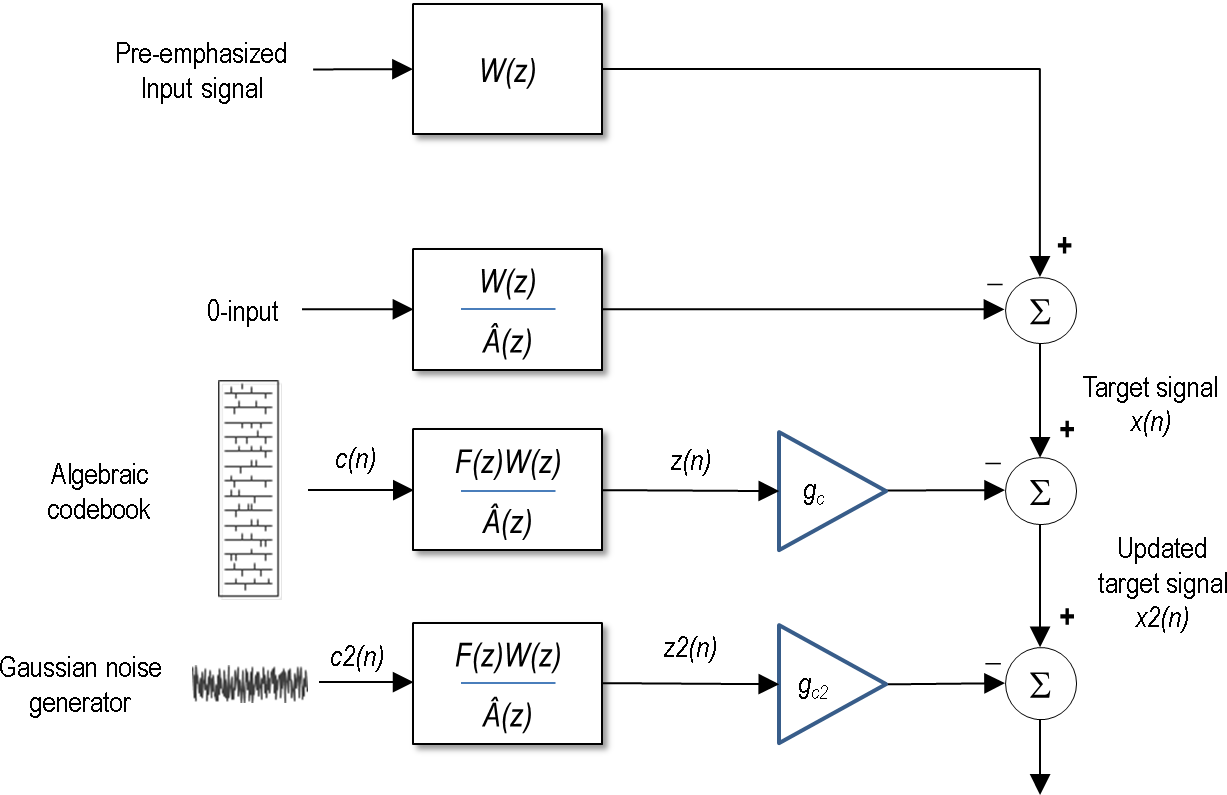Figure 39: Schematic diagram of the excitation coding in UC and IC modes at 9.6 kbps

5.2.3.4.1 Algebraic codebook

For UC mode, the adaptive pre-filter is performed similarly as in subclause 5.2.3.1.5.1. Additional the pre-filter is amended with a phase scrambling filter as follows:

(613)

For NB IC mode, the filter is designed as follows:

(614)

where is defined in subclause 5.2.3.1.5.1 with , is also defined in subclause 5.2.3.1.5.1, and and .

For WB IC mode, is defined as:

(615)

where , , and represents the tilt of following filter:

(616)

The tilt is computed as:

(617)

is bounded by [0.25 0.5] and given is by:

(618)

whereand are the energies of the scaled pitch codevector and the scaled algebraic codevector of the previous subframe, respectively.

5.2.3.4.2 Gaussian noise generation

The Gaussian noise excitation is a second excitation added to the first innovative excitation from the algebraic codebook. This second contribution is only computed and added in WB.

The Gaussian noise excitation is produced by calling three times a random generator with a uniform distribution between -1 and +1. It follows the Central Limit Theorem.

(619)

The Gaussian noisy excitation is spectrally shaped by applying the pre-filter defined in subclause 5.2.3.4.1.1.

5.2.3.4.3 Gain coding

For NB only one gain has to be quantized, the gain of the algebraic codebook . It is quantized using a 6-bit quantizer.

For WB, the two gains and are quantized jointly in each subframe, using a 7-bit vector quantizer.

In both cases the optimal algebraic codeword gain is computed as follows:

(620)

In the equation above, is the algebraic codevector filtered through the weighted synthesis filter with the pre-filter .is the filtered algebraic codevector.

The algebraic codebook excitation energy in dB, , is also computed as follows:

(621)

5.2.3.4.3.1 Innovative codebook gain coding (NB)

The algebraic codevector gain in dB is given by

(622)

is uniformly quantized between -30 dB and 90dB with the step size of 1.9dB. The quantization index is given by the integer part of

(623)

Finally, the quantized gain in dB is given by

(624)

and the quantized gain is given by

(625)

5.2.3.4.3.2 Joint gain coding (WB)

The algebraic codebook gain is quantized indirectly, using a predicted energy of algebraic codevector. The energy of residual signal in dB is calculated.

Then, average residual signal energy is calculated for the whole frame and serves as a prediction of the algebraic codevector energy. It is quantized on 4 bits once per frame. The quantized value of the predicted algebraic codevector energy is defined as

(626)

where is the 4-bit codebook for the predicted algebraic codevector energy and is the index minimizing the criterion above.

Using the predicted algebraic codevector energy, we may estimate the algebraic codebook gain as

(627)

A correction factor between the true algebraic codebook gain, , and the estimated one, , is given by

(628)

The correction factor is uniformly quantized on 5 bits between -20 dB and 20dB with the step size of 1.25dB. The quantization index is given by the integer part of

(629)

Finally, the quantized gain in dB is given by

(630)

and the quantized gain is given by

(631)

The gain of Gaussian noise excitation is quantized on 2 bits. Unlike the algebraic codeword gain, the Gaussian noise excitation gain is optimized in order to minimize the energy mismatch between the target signal and reconstructed signal. The following criterion is minimized:

(632)

where is an attenuation factor set to 1 for clean speech, where high dynamic of energy is perceptually important and set to 0.8 for noisy speech where the noise excitation is made more conservative for avoiding fluctuation in the output energy between unvoiced and non-unvoiced frames

The quantized gain is expressed as follows where the index of the optimal gain is sent on 2 bits:

(633)

5.2.3.4.4 Memory update

The update of the filter memories is performed as described in subclause 5.2.3.1.8 except that there is no adaptive codebook contribution. The Gaussian noise excitation is not taken into account for the update and for computing the next subframe signal target.

5.2.3.5 Excitation coding in GSC mode

In the GSC mode, the excitation is encoded using mixed time-domain/frequency-domain coding technique. This mode is aimed at encoding generic audio signals at low bit rates without introducing more delay than the ACELP structure requires. The GSC mode is used only at 12.8 kHz internal sampling rate, and the excitation could be encoded with 4 subframes, 2 subframes, or 1 subframe per frame depending on the bit rate or the signal type.

Figure 40 is a schematic block diagram showing the general concept of coding the excitation in the GSC mode. The speech/music selector is used to choose between coding the excitation signal in the GSC mode or the other ACELP modes described above. The selector mainly consists of the speech music classification (as described in subclause 5.1.13.5), where GSC is used in case music signals are detected. A further detector (as described in subclause 5.1.13.5.3) is used to verify if a detected music contains a temporal attack. In such a case the time domain transient coding mode is used to code only the attack.

When encoding in the GSC mode, the time-domain excitation contribution is first computed. In case of 4 subframes, the time-domain excitation consists of both adaptive codebook and fixed codebook as in ordinary ACELP. In case 1 or 2 subframes are used, the time-domain excitation consists only of the adaptive codebook contribution. Then the time-domain contribution and residual signal are both converted to the transform domain (using DCT). The transform-domain signals are used to determine a cut-off frequency (the upper band still containing significant pitch contribution). The time-domain excitation contribution is then filtered by removing the frequency content above the cut-off frequency. The filtered time-domain contribution in the frequency domain is subtracted from the frequency-domain residual signal, and difference signal is quantized in the frequency domain using PVQ. The quantized difference signal is then added to the filtered transformed time-domain excitation contribution, and the resulting signal is converted back to the time domain to obtain the total excitation signal.

The GSC mode is used for encoding audio signals at 7.2, and 13.2 kbit/s for WB inputs. It is also used to encode unvoiced active speech and some audio signal at 13.2 kbit/s in case of SWB inputs. Further, the GSC mode is used to encode inactive signals in case of NB, WB, SWB, and FB signals (in case DTX is off) at 7.2, 8 and 13.2kbit/s.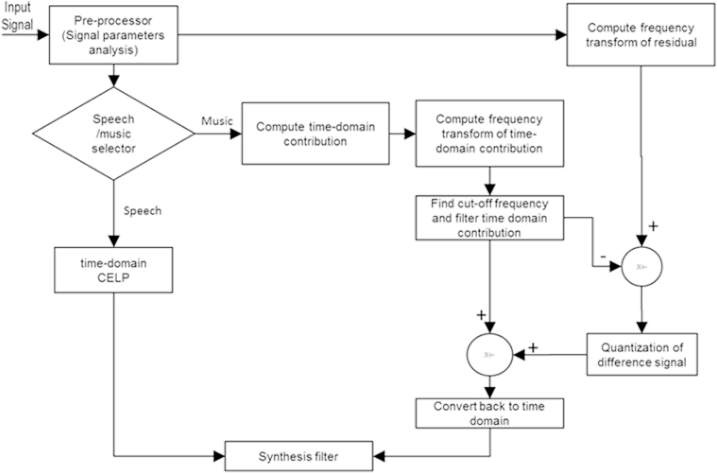Figure 41: GSC encoder overview

5.2.3.5.1 Determining the subframe length

The subframe length, or number of subframes per frame, is determined depending on the bit rate and nature of encoded signal. In case of SWB unvoiced mode at 13.2 kbit.s, 4 subframes are used. For NB and WB signals at 13.2 kbit/s, 2 subframes are used in case of inactive signals or when the high frequency dynamic range flag is 0, where is an indicator when set to 1 is indicates the presence of high frequency spectral correlation and is computed in subclause 5.1.11.2.6. Otherwise 1 subframe is used (audio signal where long-time support is needed to get better frequency resolution).

For the bit rates of 7.2 and 8 kbit/s, 1 subframe is always used (NB, WB, and SWB audio signals and inactive speech signals). The number of subframe information is encoded at 13.2 kbps with 1 bit.

5.2.3.5.2 Computing time-domain excitation contribution

For the SWB unvoiced mode at 13.2 kbit/s, 4 subframes are used and the excitation is computed similar to ACELP Generic coding mode using both adaptive and fixed codebooks (see subclause 5.2.3.1). The signal is encoded using GENERIC coding type at 7.2 kbit/s, and the remaining bits are used to encode the frequency domain contribution. The target signal for FCB search is computed without low-pass filtering of ACB excitation.

In other modes where 1 or 2 subframes are used the time-domain excitation contributions consists only of the adaptive codebook contribution. This is determined using ordinary closed-loop pitch search as in subclause 5.2.3.1.4.1.

When only 1 or 2 subframe are used, for example at 7.2 and 8 kbit/s rates, the adaptive codebook excitation and pitch gain quantization use the AUDIO coding type. The pitch found is quantized using 10 bits for the first subframe and 6 bit for the following subframe, if any. In these case, he pitch gain is quantized using a 4 bits vector quantizer.

The total excitation is finally constructed based on both ACB and FCB at 13.2 UC mode or only ACB contribution for other modes using a total between 14 and 24 bits in case of 1 or 2 subframe up to 106 bits for the 4 subframe case..

5.2.3.5.3 Frequency transform of residual and time-domain excitation contribution

In the frequency-domain coding of the mixed time-domain / frequency-domain GSC mode, the residual signal and the time-domain excitation contribution are transformed to frequency domain. The time-to-frequency transform is performed using a 256-point Type IV discrete cosine transform (DCTIV) giving a resolution of 25 Hz at the internal sampling frequency of 12.8 kHz.

The DCTIV, , of a signal of length is defined by the following equation:

(634)

Here and refers to either residual signal or time-domain excitation contribution with DCT output corresponding to frequency transformed signals and , respectively.

5.2.3.5.3.1 eDCT for DCTIV

The efficient eDCT is built upon a discrete cosine transform type IV (DCTIV) but the eDCT requires less storage and has lower complexity.

The DCTIV formula in above subclause can be rewritten as:

(635)

where the values are given by

(636)

and , , and .

Hence, the eDCT is computed using a Fast Fourier Transform (FFT) of points on the pre-rotated data :

A complex DFT with length is applied to the rotated data :

(637)

Here, when , a simple power-2 DFT is not suitable, so it is implemented with the following low complexity 2-dimensional () DFT, where and are coprime factors.

To reduce complexity, an address table is introduced. It can be calculated by:

(638)

where , are coprime and satisfy the condition .

Here, . The address table is stored for low complexity 2-dimentional DFT, and is used to indicate which samples are used for -point DFT or -point DFT following:

a) Applying -point DFT to for times based on the address table .

The input data to the i-th ()-point DFT is found by seeking their addresses stored in the address table . For the i-th -point DFT, the addresses of the input data are the continuous elements starting from the element in table . For every time of -point DFT, the resulting data need to be applied a circular shift with a step of , where is the re‑ordered index, which satisfies .

The output of step a) is:

(639)

For the i-th ()-point DFT, the addresses of the input data are the continuous elements starting from , and the results are circular shifted with . Here is an example of circular shift, the original vector is , the new vector with 2 circular shift is .

b) Applying -point DFT to for times based on the address table .

The input data to the i-th ()-point DFT is found by seeking their addresses stored in the address table . For the i-th -point DFT, the addresses of the input data are the elements starting from the i-th element in table , each of which separated by a step of . For every time of -point DFT, the resulting data need to be applied a circular shift with a step of . is the re-ordered index, which satisfies .

The output of step b) is:

(640)

For the i-th ()-point DFT, the addresses of the input data are the continuous elements starting from , each of which is separated by a step of , and the results are circular shifted with . Finally, the coefficients are output according to the stored address corresponding to the address table .

5.2.3.5.4 Computing energy dynamics of transformed residual and quantization of noise level

The DCT of the residual is divided into 16 bands (0 to 15) of length 16 bins. For bands 7 to 14, the energy dynamic per band is computed as the square of the maximum value divided by the average value per band, scaled by a factor 10. Then the average value over the 8 band (from 7 to 14) is computed.

A long-term dynamic is updated as

(641)

is quantized with 8 levels in the rage 50-82 (50, 54, 58, 62, 66, 70, 74, 78) with quantization index from 0 to 7.

The noise level is computed as

For the bit rates of 7.2 and 8 kbit/s, the noise level is low limited to 12. Thus only values 12, 13, 14, 15 are permitted and is quantized with 2 bits). For UC SWB mode at 13.2 kbit/s is set to 15, otherwise is quantized with 3 bits (values 8 to 15).

5.2.3.5.6 Find and encode the cut-off frequency

The cut-off frequency consists of the last band with significant pitch contribution (the frequency after which coding improvement brought by the time-domain excitation contribution becomes too low to be valuable). Finding the cut-off frequency starts by computing the normalized cross-correlation for each frequency band between the frequency-transformed LP residual and the frequency-transformed time-domain excitation contribution . The 256-sample DCT spectrum is divided into the 16 bands with the following number of frequency bins per band

(642)

with cumulative frequency bins per band

(643)

The last frequency included in each of the 16 frequency bands are defined in Hz as:

(644)

The normalized correlation per band is defined as

(645)

Where and .

The cross-correlation vector is then smoothed between the different frequency bands using the following relation

(646)

where , and

The average of the smoothed cross-correlation vector is computed over the first 13 bands (representing 5575 Hz). It is then limited to a minimum value of 0.5 normalised between 0 and 1.

A first estimate of the cut-off frequency is obtained by finding the last frequency of a frequency band which minimizes the difference between the last frequency of a frequency band and the normalized average of the smoothed cross-correlation vector multiplied by the width of the spectrum of the input sound signal. That is

(647)

and the first estimate of the cut-off frequency is given by

(648)

where Hz.

At 7.2 and 8 kbit/s, where the normalized average is never really high, or to artificially increase the value of to give a little more weight to the time domain contribution, the value of is upscaled with a factor of 2.

The 8th pitch harmonic is computed from the minimum or lowest pitch lag value of the time-domain excitation contribution of all sub-frames, and the frequency band containing the 8th harmonic is determined. The final cut-off frequency is given by the higher value between the first estimate of the cut-off frequency and the last frequency of the frequency band in which the 8th harmonic is located .

The cut-off frequency is quantized with a maximum of 4 bits using the values {0, 1175, 1575, 1975, 2775, 3175, 3575, 3975, 4375, 4775, 5175, 5575, 6375}.

Some hangover is added to stabilize the decision and prevent the cut-off frequency to switch between 0 (meaning no temporal contribution) and something else too often. First for the temporal contribution to be allowed, the average normalized correlation and the long-term correlation as computed in subclause 5.1.13.5.3, the long term average pitch gain of the GSC temporal contribution and the last value of the cut-off frequency are compared to some threshold to decide if it is allowed to remove all the temporal contribution (cut-off frequency would be 0). In addition a hangover logic is used to diminish any undesired switching to a complete frequency model where the cut-off frequency would be 0.

For the lowest bitrate, 7.2 and 8.0 kbit/s, only 1 bit is used to send the cut-off frequency information when the coding mode is INACTIVE otherwise, the cut-off frequency is considered as greater than 0 (meaning the temporal contribution is used) and the length of the contribution is deduced from the pitch information. At 13 kbps, 4 bits are used to send the cut-off frequency allowing all the possible cut-off frequency values.

Once the cut-off frequency is determined, the transform of the time-domain excitation contribution is filtered in the frequency domain by zeroing the frequency bins situated above the cut-off frequency supplemented with a smooth transition region. The transition region is situated above the cut-off frequency and below the zeroed bins, and it allows for a smooth spectral transition between the unchanged spectrum below and the zeroed bins in higher frequencies.

5.2.3.5.7 Band energy computation and quantization

The filtered time-domain contribution in the frequency domain is subtracted from the frequency-domain residual signal , and the resulting difference signal in the frequency domainis quantized with the PVQ. Before the quantization is done, some gains per frequency band , as defined above, are computed and quantized using a split VQ. First the gain per band on the difference signal is computed as :

(649)

where and are defined in subclause 5.2.3.5.6.

In case of NB content, only the first 10 bands are quantized using a split VQ. For other bandwidth, the number of band quantized depends on the bitrate. At low bit rate only 12 bands are quantized, being the band 0 to 8 plus the bands 10, 12 and 14. The band 9, 11, 13 and 15 being interpolated based on the quantized bands 8, 10, 12, and 14. The codebook used for the vector quantization are different depending of the bitrate and the bandwidth of the input signal giving a total 4 different set of codebooks.

In all cases, prior to the vector quantitation of the bands, the average gain of all the bands is subtract from the bands and vector quantized as well using 6 bits. In total between 21 and 26 bits are used to get the gain per band quantized depending of the bitrate.

5.2.3.5.8 PVQ Bit allocation

The PVQ is a coding technic that is flexible in its bit allocation. To decide where bits should be allocated inside the difference spectrum to quantize, some parameters are analyse as the bitrate, the cut-off frequency, the noise level, the coding mode (INACTIVE, AUDIO or active UC), the bit budget available and the bandwidth.

First, only a subset of bands will be sent to the PVQ for quantization. The minimum number of band is 5 out of 16. To determine the number of band, a first criteria is the bit rate, a second criteria is the cut-off frequency and another criteria is noise level. When the number of band is decided, a minimum amount of bit is spread over the number of band decided with an emphasis on the low frequencies. If some bits remain after the minimum bit allocation, then the remaining bits are split among the bands. When the number of bands and its bit allocation are found, the bands are picked from the initial spectrum of the difference signal based on the quantized gain of this band. The 5 first bands are always sent to the PVQ, the choice of the other bands on the energy associated to that band and the high frequency flag indicator.

5.2.3.5.9 Quantization of difference signal

Once the bit allocation the number of band to quantize and their position in the spectrum is defined, a new vector is concatenated containing all the chosen bands. The values are then passed to the PVQ for quantization to obtain the quantized difference spectrum. The PVQ quantization scheme is described in subclause 5.3.4.2.7.

5.2.3.5.10 Spectral dynamic and noise filling

After the quantization by the PVQ, some band are empty and many more bins are zeroed due to the low inherent to the GSC technology available. To make the frequency model as robust as possible on speech like content, the spectral dynamic is revised and some noise filling is added to the difference spectrum.

For INACTIVE content below 13.2 kbit/s, the quantized spectrum above 1.6kHz is multiplied by a factor of 0.15. For INACTIVE content at 13.2 kHz, the quantized spectrum above 2.0kHz is multiplied by a factor of 0.25. Otherwise the scaling factor for the spectral dynamic and the frequency bin where the scaling of the spectral dynamic is applied is computed as follow:

(650)

and

(651)

Furthermore, for frequencies above 3.2 kHz, the spectral dynamic is limited to an amplitude of ±1 for bitrate below 13.2 kbit/s and to ± 1.5 otherwise.

This scaling is then applied to the quantized difference spectrum, to obtain its scaled version.

A noise filling is then applied to the whole difference spectrum. The noise level added is based on the bitrate, the coding mode and the spectral dynamic to obtain the scaled difference spectrum with noiseon which the gain will be applied on.

5.2.3.5.11 Quantized gain addition, temporal and frequency contributions combination

Once dynamic of the quantized difference spectrum has been scaled and the noise fill has be performed, the gain of each bands is computed exactly as in subclause 5.2.3.5.7 to get gain of the quantized spectrum. The gain per band to apply consists as:

(652)

This gain is applied to both the scaled difference spectrum with noise and the scaled difference spectrumand both vectors are added to the temporal contribution to get two different spectral representation of the quantized excitation in the frequency domain, one with noise fill and the other without as shown below.

(653)

and

(654)

5.2.3.5.12 Specifics for wideband 8kbps

The available bits are allocated to the bands of the frequency excitation signal according to the bit allocation algorithm as described in subclause 5.2.3.5.8, where the frequency excitation signal is the output of DCTIV as described in subclause 5.2.3.5.3. If the index of the highest frequency band with bit allocation is more than a given threshold, the bit allocation for the frequency excitation bands will be adjusted: Decrease the number of the allocated bits of the bands with more bits, and increase the number of the allocated bits of the band and the bands near to . And then, encode the frequency excitation signal with the allocated bits, where the given threshold is determined by the available bits and the resolution of the frequency excitation signal.

The details are described as follows:

1. Allocate most of the available bits to the 5 lower frequency bands by the pre-determined bit allocation table;
2. Allocate the remaining bits to those bands excluding the lower frequency 5 bands which have the largest band energy, if there are remaining bits after the first step;
3. Search the index of the highest frequency band with bit allocation.

If the index of the highest frequency band with bit allocation is more than a given threshold, the bit allocation for the frequency excitation bands will be adjusted:

1. Allocate bits to some more bands whose index is above . The number of the newly bit allocated bands is determined by the noise leveland the coding mode.
2. For the newly bit allocated bands, allocate 5 bits to each band. If the number of the newly bit allocated bands, allocate 1 more bit to each band whose index starts from 4 to .
3. The total number of newly allocated bits is . is obtained by decreasing the number of the bits allocated to the 4 lower frequency bands.

Otherwise, the original number of allocated bits to each band is not changed.

Finally, quantize and encode the frequency excitation signal according to the allocated bits.

Then, reconstruct the frequency excitation signal based on the quantized parameters. The reconstructed frequency excitation signal is corresponding to the decoded frequency excitation signal in decoder.

For the reconstructed frequency excitation signal, if the index of the highest frequency band with bit allocation is more than a given threshold, or there is the temporal contribution in the reconstructed frequency excitation signal, the frequency excitation signal above will be reconstructed by the reconstructed frequency excitation signal; otherwise, the frequency excitation signal above will be reconstructed by noise filling.

The detailed descriptions are as follows:

When the coding mode of previous frame is AC mode, if the last sub-band index of bit allocation is larger than or there is the temporal contribution in the reconstructed frequency excitation signal, the BWE flag is set to 1. It should be noted that the BWE flag is initialized to 0 and calculated for every frame. is then refined by:

(655)

If , the frequency excitation signal below will be reconstructed as described in subclause 5.2.3.5.11, and the frequency excitation signal above will be reconstructed as follows:

(656)

And then the frequency excitation signal is scaled by the quantized gains to obtain the scaled frequency excitation signal .

When the energy ratio between the current frame and the previous frame is in the range (0.5, 2), for any band with index range is [4, 9], if the band is bit allocated in the current frame or in the previous frame, the coefficients in the band are smoothed by weighting the coefficients of the current frame and the previous frame.

For the scaled frequency excitation signal above , estimate the position of the formant by LSF parameters. If the magnitudes of the coefficients near to the formant are larger than a threshold, the magnitudes are decreased to improve the perceptual quality.

Otherwise, If , the un-quantized coefficients, i.e. un-decoded coefficients at the decoder side will be reconstructed by noise filling as described in subclause 5.2.3.5.10.

5.2.3.5.13 Inverse DCT

After the gain has been applied and the combination in the frequency domain done, both frequency representations of the coded excitation are convert back to time domain using the exact same DCT as in subclause 5.2.3.5.3. The inverse transform is performed to get the quantized excitation which is the temporal representation of and which is the temporal representation of. will be used to update the TDBWE while is used to update the internal CELP state as the adaptive codebook memory.

5.2.3.5.14 Remove pre-echo in case of onset detection

Compute the energy of the excitation over each 4 samples using a 4-sample sliding window, and find the more energetic section to determine a possible attack (onset). If the attack is larger than the previous frame energy plus 6 dB, the algorithm finds the energy before the attack (excluding the section where the attack has been detected) and it scales it to the level of the previous frame energy plus 6dB.